# MLP, Numpy, TF2 – performance issues – Step II – bias neurons, F- or C- contiguous arrays and performance

Welcome back, my friends of MLP coding. In the last article we gave the code developed in the article series

A simple Python program for an ANN to cover the MNIST dataset – I – a starting point

a first performance boost by two simple measures:

• We set all major arrays to the „numpy.float32“ data type instead of the default „float64“.
• In addition we eliminated superfluous parts of the backward [BW] propagation between the first hidden layer and the input layer.

This brought us already down to around

11 secs for 35 epochs on the MNIST dataset, a batch-size of 500 and an accuracy around 99 % on the training set

This was close to what Keras (and TF2) delivered for the same batch size. It marks the baseline for further performance improvements of our MLP code.

Can we get better than 11 secs for 35 epochs? The answer is: Yes, we can – but only in small steps. So, do not expect any gigantic performance leaps for the training loop itself. But, there was and is also our observation that there is no significant acceleration with growing batch sizes over 1000 – but with Keras we saw such an acceleration.

In this article I shall shortly discuss why we should care about big batch sizes – at least in combination with FW-propagation. Afterwards I want to draw your attention to a specific code segment of our MLP. We shall see that an astonishingly simple array operation dominates the CPU time of our straight forward coded FW propagation. Especially for big batch sizes!

Actually, it is an operation I would never have guessed to be such an an obstacle to efficiency if somebody had asked me. As a naive Python beginner I had to learn that the arrangement of arrays in the computer’s memory sometimes have an impact – especially when big arrays are involved. To get to this generally useful insight we will have to invest some effort into performance tests of some specific Numpy operations on arrays. The results give us some options for possible performance improvements; but in the end we shall circumvent the impediment all together.

The discussion will indicate that we should change our treatment of bias neurons fundamentally. We shall only go a preliminary step in this direction. This step will give us already a 15% improvement regarding the training time. But even more important, it will reward us with a significant improvement – by a factor > 2.5 – with respect to the FW-propagation of the complete training and test data sets, i.e. for the FW-propagation of „batches“ with big sizes (60000 or 10000 samples).

„np.“ abbreviates the „Numpy“ library below. I shall sometimes speak of our 2-dimensional Numpy „arrays“ as „matrices“ in an almost synonymous way. See, however, one of the links at the bottom of the article for the subtle differences of related data types. For the time being we can safely ignore the mathematical differences between matrices, stacks of matrices and tensors. But we should have a clear understanding of the profound difference between the „*„-operation and the „np.dot()„-operation on 2-dimensional arrays.

# Why are big batch sizes relevant?

There are several reasons why we should care about an efficient treatment of big batches. I name a few, only:

• Numpy operations on bigger matrices may become more efficient on systems with multiple CPUs, CPU cores or multiple GPUs.
• Big batch sizes together with a relatively small learning rate will lead to a smoother descent path on the cost hyperplane. Could become important in some intricate real life scenarios beyond MNIST.
• We should test the achieved accuracy on evaluation and test datasets during training. This data sets may have a much bigger size than the training batches.

The last point addresses the problem of overfitting: We may approach a minimum of the loss function of the training data set, but may leave the minimum of the cost function (and of related errors) of the test data set at some point. Therefore, we should check the accuracy on evaluation and test data sets already during the training phase. This requires the FW-propagation of such sets – preferably in one sweep. I.e. we talk about the propagation of really big batches with 10000 samples or more.

How do we measure the accuracy? Regarding the training set we gather averaged errors of batches during the training run and determine the related accuracy at the end of every printout period via an average over all batches: The average is taken over the absolute values of the difference between the sigmoidal output and the one-hot encoded target values of the batch samples. Note that this will give us slightly different values than tests where Numpy.argmax() is applied to the output first.

We can verify the accuracy also on the complete training and test data sets. Often we will do so after each and every epoch. Then we involve argmax(), by the way to get numbers in terms of correctly classified samples.

We saw that the forward [FW] propagation of the complete training data set „X_train“ in one sweep requires a substantial (!) amount of CPU time in the present state of our code. When we perform such a test at each and every epoch on the training set the pure training time is prolonged by roughly a factor 1.75. As said: In real live scenarios we would rather or in addition perform full accuracy tests on prepared evaluation and test data sets – but they are big „batches“ as well.

So, one relevant question is: Can we reduce the time required for a forward [FW] propagation of complete training and test data sets in one vectorized sweep?

# Which operation dominates the CPU time of our present MLP forward propagation?

The present code for the FW-propagation of a mini-batch through my MLP comprises the following statements – enriched below by some lines to measure the required CPU-time:

```
''' -- Method to handle FW propagation for a mini-batch --'''
def _fw_propagation(self, li_Z_in, li_A_out):
'''
Parameter:
li_Z_in :   list of input values at all layers  - li_Z_in is already filled -
other elemens to to be filled during FW-propagation
li_A_out:   list of output values at all layers - to be filled during FW-propagation
'''
# index range for all layers
#    Note that we count from 0 (0=>L0) to E L(=>E) /
#    Careful: during BW-propagation we need a clear indexing of the lists filled during FW-propagation
ilayer = range(0, self._n_total_layers-1)

# propagation loop
# ***************
for il in ilayer:

# Step 1: Take input of last layer and apply activation function
# ******
ts=time.perf_counter()
if il == 0:
A_out_il = li_Z_in[il] # L0: activation function is identity !!!
else:
A_out_il = self._act_func( li_Z_in[il] ) # use defined activation function (e.g. sigmoid)
te=time.perf_counter(); ta = te - ts; print("\nta = ", ta, " shape = ", A_out_il.shape, " type = ", A_out_il.dtype, " A_out flags = ", A_out_il.flags)

# Step 2: Add bias node
# ******
ts=time.perf_counter()
A_out_il = self._
li_A_out[il] = A_out_il
te=time.perf_counter(); tb = te - ts; print("tb = ", tb, " shape = ", A_out_il.shape, " type = ", A_out_il.dtype)

# Step 3: Propagate by matrix operation
# ******
ts=time.perf_counter()
Z_in_ilp1 = np.dot(self._li_w[il], A_out_il)
li_Z_in[il+1] = Z_in_ilp1
te=time.perf_counter(); tc = te - ts; print("tc = ", tc, " shape = ", li_Z_in[il+1].shape, " type = ", li_Z_in[il+1].dtype)

# treatment of the last layer
# ***************************
ts=time.perf_counter()
il = il + 1
A_out_il = self._out_func( li_Z_in[il] ) # use the defined output function (e.g. sigmoid)
li_A_out[il] = A_out_il
te=time.perf_counter(); tf = te - ts; print("\ntf = ", tf)

return None

```

The attentive reader notices that I also included statements to print out information about the shape and so called „flags“ of the involved arrays.

I give you some typical CPU times for the MNIST dataset first. Characteristics of the test runs were:

• data were taken during the first two epochs;
• the batch-size was 10000; i.e. we processed 6 batches per epoch;
• „ta, tb, tc, tf“ are representative data for a single batch comprising 10000 MNIST samples.

Averaged timing results for such batches are:

```Layer L0
ta =  2.6999987312592566e-07
tb =  0.013209896002081223
tc =  0.004847299001994543
Layer L1
ta =  0.005858420001459308
tb =  0.0005839099976583384
tc =  0.00040631899901200086
Layer L2
ta =  0.0025550600003043655
tb =  0.00026626299950294197
tc =  0.00022965300013311207
Layer3
tf =  0.0008438359982392285
```

Such CPU time data vary of course a bit (2%) with the background activity on my machine and with the present batch, but the basic message remains the same. When I first saw it I could not believe it:

Adding a bias-neuron to the input layer obviously dominated the CPU-consumption during forward propagation. Not the matrix multiplication at the input layer L0!

I should add at this point that the problem increases with growing batch size! (We shall see this later in elementary test, too). This means that propagating the complete training or test dataset for accuracy check at each epoch will cost us an enormous amount of CPU time – as we have indeed seen in the last article. Performing a full propagation for an accuracy test at the end of each and every epoch increased the total CPU time roughly by a factor of 1.68 (19 sec vs. 11.33 secs for 35 epochs; see the last article).

# Adding a row of constant input values of bias neurons

I first wanted to know, of course, whether my specific method of adding a bias neuron to the A-output matrix at each layer really was so expensive. My naive approach – following a suggestion in a book of S. Rashka, by the way – was:

```def add_bias_neuron_to_layer(A, how='column'):
if how == 'column':
A_new = np.ones((A.shape, A.shape+1), dtype=np.float32)
A_new[:, 1:] = A
elif how == 'row':
A_new = np.ones((A.shape+1, A.shape), dtype=np.float32)
A_new[1:, :] = A
return A_new
```

What we do here is to create a new array which is bigger by one row and fit the original array into it. Seemed to be a clever approach at the time of coding (and actually it is faster than using np.vstack or np.hstack). The operation is different from directly adding a row to the existing input array explicitly, but it still requires a lot of row operations.

As we have seen I call this function in „_fw_
propagation()“ by

```A_out_il = self._add_bias_neuron_to_layer(A_out_il, 'row')
```

„A_out_il“ is the transposition of a slice of the original X_train array. The slice in our test case for MNIST had a shape of (10000, 784).
This means that we talk about a matrix with shape (784, 10000) in the case of the MNIST dataset before adding the bias neuron and a shape of (785, 10000) after. I.e. we add a row with 10000 constant entries at the beginning of our transposed slice. Note also that the function returns a new array in memory.

Thus, our approach contains two possibly costly operations. Why did we do such a strange thing in the first place?

Well, when we coded the MLP it seemed to be a good idea to include the fact that we have bias neurons directly in the definition of the weight matrices and their shapes. So, we need(ed) to fit our input matrices at the layers to the defined shape of the weight matrices. As we see it now, this is a questionable strategy regarding performance. But, well, let us not attack something at the very center of the MLP code for all layers (except the output layer) at this point in time. We shall do this in a forthcoming article.

# A factor of 3 ??

To understand my performance problem a bit better, I did the following test in a Jupyter cell:

```''' Method to add values for a bias neuron to A_out  all with C-cont. arrays '''
if how == 'column':
A_new = np.ones((A.shape, A.shape+1), dtype=np.float32)
A_new[:, 1:] = A
elif how == 'row':
A_new = np.ones((A.shape+1, A.shape), dtype=np.float32)
A_new[1:, :] = A
return A_new
input_shape =(784, 10000)
ay_inpC = np.array(np.random.random_sample(input_shape)*2.0, dtype=np.float32)
tx = time.perf_counter()
li_A.append(ay_inpCb)
ty = time.perf_counter(); t_biasC = ty - tx;
print("\n bias time = ", "%10.8f"%t_biasC)
print("shape_biased = ", ay_inpCb.shape)
```

to get:

``` bias time  =  0.00423444
```

Same batch-size, but substantially faster – by roughly a factor of 3! – compared to what my MLP code delivered. Actually the timing data varied a bit between 0.038 and 0.045 (with an average at 0.0042) when repeating the run. To exclude any problems with calling the function from within a Python class I repeated the same test inside the class „MyANN“ during FW-propagation – with the same result (as it should be; see the first link at the end of this article).

So: Applying one and the same function on a randomly filled array was much faster than applying it on my Numpy (input) array „A_out_il“ (with the same shape). ????

# C- and F-contiguous arrays

It took me a while to find the reason: „A_out_il“ is the result of a matrix transposition. In Numpy this corresponds to a certain view on the original array data – but this still has major consequences for the handling of the data:

A 2 dimensional array or matrix is an ordered addressable sequence of data in the computer’s memory. Now, if you yourself had to program an array representation in memory on a basic level you would – due to performance reasons – make a choice whether you arrange data row-wise or column-wise. And you would program functions for array-operations with your chosen „order“ in mind!

Actually, if you google a bit you find that the two ways of arranging array or matrix data are both well established. In connection with Numpy we speak of either a C-contiguous order or a F-contiguous order of the array data. In the first case (C) data are stored and addressed row by row and can be read efficiently this way, in the other (F) case data are arranged
column by column. By the way: The „C“ refers to the C-language, the „F“ to Fortran.

On a Linux system Numpy normally creates and operates with C-contiguous arrays – except when you ask Numpy explicitly to work differently. Quite many array related functions, therefore, have a parameter „order“, which you can set to either ‚C‘ or ‚F‘.

Now, let us assume that we have a C-contiguous array. What happens when we transpose it – or look at it in a transposed way? Well, logically it then becomes F-contiguous! Then our „A_out_il“ would be seen as F-contiguous. Could this in turn have an impact on performance? Well, I create „A_out_il“ in method „_handle_mini_batch()“ of my MyANN-class via

```        # Step 0: List of indices for data records in the present mini-batch
# ******
ay_idx_batch = self._ay_mini_batches[num_batch]

# Step 1: Special preparation of the Z-input to the MLP's input Layer L0
# ******
# Layer L0: Fill in the input vector for the ANN's input layer L0
li_Z_in_layer = self._X_train[ay_idx_batch] # numpy arrays can be indexed by an array of integers
li_Z_in_layer  = li_Z_in_layer.T
...
...
```

Hm, pretty simple. But then, what happens if we perform our rather special adding of the bias-neuron row-wise, as we logically are forced to? Remember, the array originally had a shape of (10000, 784) and after transposing a shape of (784, 10000), i.e. the columns then represent the samples of the mini-batch. Well, instead of inserting a row of 10000 data contiguously into memory in one swipe into a C-contiguous array we must hop to the end of each contiguous column of the F-contiguous array „A_out_il“ in memory and add one element there. Even if you would optimize it there are many more addresses and steps involved. Can’t become efficient ….

How can we see, which order an array or view onto it follows? We just have to print its „flags„. And I indeed got:

```flags li_Z_in =
C_CONTIGUOUS : False
F_CONTIGUOUS : True
OWNDATA : False
WRITEABLE : True
ALIGNED : True
WRITEBACKIFCOPY : False
UPDATEIFCOPY : False
```

Let us extend the tests of our function in the Jupyter cell in the following way to cover a variety of options related to our method of adding bias neurons:

```
# The bias neuron problem
# ************************
import numpy as np
import scipy
from scipy.special import expit
import time

''' Method to add values for a bias neuron to A_out - by creating a new C-cont. array '''
if how == 'column':
A_new = np.ones((A.shape, A.shape+1), dtype=np.float32)
A_new[:, 1:] = A
elif how == 'row':
A_new = np.ones((A.shape+1, A.shape), dtype=np.float32)
A_new[1:, :] = A
return A_new

''' Method to add values for a bias neuron to A_out - by creating a new F-cont. array '''
if how == 'column':
A_new = np.ones((A.shape, A.shape+1), order='F', dtype=np.float32)
A_new[:, 1:] = A
elif how == 'row':
A_new = np.ones((A.shape+1, A.shape), order='F', dtype=np.float32)
A_new[1:, :] = A
return A_new

rg_j = range(50)

li_A = []

t_1 = 0.0; t_2 = 0.0;
t_3 = 0.0; t_4 = 0.0;
t_5 = 0.0; t_6 = 0.0;
t_7 = 0.0; t_8 = 0.0;

# two types of input shapes
input_shape1 =(784, 10000)
input_shape2 =(10000, 784)

for j in rg_j:

# For test 1: C-cont. array with shape (784, 10000)
# in a MLP programm delivering X_train as (
10000, 784) we would have to (re-)create it
# explicitly with the C-order (np.copy or np.asarray)
ay_inpC = np.array(np.random.random_sample(input_shape1)*2.0, order='C', dtype=np.float32)

# For test 2: C-cont. array with shape (10000, 784) as it typically is given by a slice of the
# original X_train
ay_inpC2 = np.array(np.random.random_sample(input_shape2)*2.0, order='C', dtype=np.float32)

# For tests 3 and 4: transposition - this corresponds to the MLP code
ay_inpF = ay_inpC2.T

# For test 5: The original X_train or mini-batch data are somehow given in F-cont.form,
# then inpF3 below would hopefully be in C-cont. form
ay_inpF2 = np.array(np.random.random_sample(input_shape2)*2.0, order='F', dtype=np.float32)

# For test 6
ay_inpF3 = ay_inpF2.T

# Test 1:  C-cont. input to add_bias_neuron_to_layer_C - with a shape that fits already
# ******
tx = time.perf_counter()
li_A.append(ay_Cb)
ty = time.perf_counter(); t_1 += ty - tx;

# Test 2:  Standard C-cont. input to add_bias_neuron_to_layer_C - but col.-operation due to shape
# ******
tx = time.perf_counter()
li_A.append(ay_C2b)
ty = time.perf_counter(); t_2 += ty - tx;

# Test 3:  F-cont. input to add_bias_neuron_to_layer_C (!) - but row-operation due to shape
# ******   will give us a C-cont. output array which later is used in np.dot() on the left side
tx = time.perf_counter()
li_A.append(ay_C3b)
ty = time.perf_counter(); t_3 += ty - tx;

# Test 4:  F-cont. input to add_bias_neuron_to_layer_F (!) - but row-operation due to shape
# ******   will give us a F-cont. output array which later is used in np.dot() on the left side
tx = time.perf_counter()
li_A.append(ay_F4b)
ty = time.perf_counter(); t_4 += ty - tx;

# Test 5:  F-cont. input to add_bias_neuron_to_layer_F (!) - but col-operation due to shape
# ******   will give us a F-cont. output array with wrong shape for weight matrix
tx = time.perf_counter()
li_A.append(ay_F5b)
ty = time.perf_counter(); t_5 += ty - tx;

# Test 6:  C-cont. input to add_bias_neuron_to_layer_C (!) -  row-operation due to shape
# ******   will give us a C-cont. output array with wrong shape for weight matrix
tx = time.perf_counter()
li_A.append(ay_C6b)
ty = time.perf_counter(); t_6 += ty - tx;

# Test 7:  C-cont. input to add_bias_neuron_to_layer_F (!) -  row-operation due to shape
# ******   will give us a F-cont. output array with wrong shape for weight matrix
tx = time.perf_counter()
li_A.append(ay_F7b)
ty = time.perf_counter(); t_7 += ty - tx;

print("\nTest 1: nbias time C-cont./row with add_.._C() => ", "%10.8f"%t_1)
print("shape_ay_Cb = ", ay_Cb.shape, " flags = \n", ay_Cb.flags)

print("\nTest 2: nbias time C-cont./col with add_.._C() => ", "%10.8f"%t_2)
print("shape of ay_C2b = ", ay_C2b.shape, " flags = \n", ay_C2b.flags)

print("\nTest 3: nbias time F-cont./row with add_.._C() => ", "%10.8f"%t_3)
print("shape of ay_C3b = ", ay_C3b.shape, " flags = \n", ay_C3b.flags)

print("\nTest 4: nbias time F-cont./row with add_.._F() => ", "%10.8f"%t_4)
print("shape of ay_F4b = ", ay_F4b.shape, " flags = \n", ay_F4b.flags)

print("\nTest 5: nbias time F-cont./col
print("shape of ay_F5b = ", ay_F5b.shape, " flags = \n", ay_F5b.flags)

print("\nTest 6: nbias time C-cont./row with add_.._C() => ", "%10.8f"%t_6)
print("shape of ay_C6b = ", ay_C6b.shape, " flags = \n", ay_C6b.flags)

print("\nTest 7: nbias time C-cont./col with add_.._F() => ", "%10.8f"%t_7)
print("shape of ay_F7b = ", ay_F7b.shape, " flags = \n", ay_F7b.flags)
```

You noticed that I defined two different ways of creating the bigger array into which we place the original one.

Results are:

```
Test 1: bias time C-cont./row with add_.._C() =>  0.20854935
shape_ay_Cb =  (785, 10000)  flags =
C_CONTIGUOUS : True
F_CONTIGUOUS : False
OWNDATA : True
WRITEABLE : True
ALIGNED : True
WRITEBACKIFCOPY : False
UPDATEIFCOPY : False

Test 2: bias time C-cont./col with add_.._C() =>  0.25661559
shape of ay_C2b =  (10000, 785)  flags =
C_CONTIGUOUS : True
F_CONTIGUOUS : False
OWNDATA : True
WRITEABLE : True
ALIGNED : True
WRITEBACKIFCOPY : False
UPDATEIFCOPY : False

Test 3: bias time F-cont./row with add_.._C() =>  0.67718296
shape of ay_C3b =  (785, 10000)  flags =
C_CONTIGUOUS : True
F_CONTIGUOUS : False
OWNDATA : True
WRITEABLE : True
ALIGNED : True
WRITEBACKIFCOPY : False
UPDATEIFCOPY : False

Test 4: nbias time F-cont./row with add_.._F() =>  0.25958392
shape of ay_F4b =  (785, 10000)  flags =
C_CONTIGUOUS : False
F_CONTIGUOUS : True
OWNDATA : True
WRITEABLE : True
ALIGNED : True
WRITEBACKIFCOPY : False
UPDATEIFCOPY : False

Test 5: nbias time F-cont./col with add_.._F() =>  0.20990409
shape of ay_F5b =  (10000, 785)  flags =
C_CONTIGUOUS : False
F_CONTIGUOUS : True
OWNDATA : True
WRITEABLE : True
ALIGNED : True
WRITEBACKIFCOPY : False
UPDATEIFCOPY : False

Test 6: nbias time C-cont./row with add_.._C() =>  0.22129941
shape of ay_C6b =  (785, 10000)  flags =
C_CONTIGUOUS : True
F_CONTIGUOUS : False
OWNDATA : True
WRITEABLE : True
ALIGNED : True
WRITEBACKIFCOPY : False
UPDATEIFCOPY : False

Test 7: nbias time C-cont./col with add_.._F() =>  0.67642328
shape of ay_F7b =  (10000, 785)  flags =
C_CONTIGUOUS : False
F_CONTIGUOUS : True
OWNDATA : True
WRITEABLE : True
ALIGNED : True
WRITEBACKIFCOPY : False
UPDATEIFCOPY : False

```

These results

• confirm that it is a bad idea to place a F-contiguous array or (F-contiguous view on an array) into a C-contiguous one the way we presently do it;
• confirm that we should at least create the surrounding array with the same order as the input array, which we place into it.

The best combinations are

1. either to put an original C-contiguous array with fitting shape into a C-contiguous one with one more row,
2. or to place an original F-contiguous array with suitable shape into a F-contiguous one with one more column.

By the way: Some systematic tests also showed that the time difference between the first and the third operation grows with batch size:

```bs = 60000, rep. = 30   => t1=0.70, t3=2.91, fact=4.16
bs = 50000, rep. = 30   => t1=0.58, t3=2.34, fact=4.03
bs = 40000, rep. = 50   => t1=0.78, t3=3.07, fact=3.91
bs = 30000, rep. = 50   => t1=0.60, t3=2.21, fact=3.68
bs = 20000, rep. = 60   => t1=0.49, t3=1.63, fact=3.35
bs = 10000, rep. = 60   => t1=0.26, t3=0.82, fact=3.20
bs =  5000, rep. = 60   => t1=0.
11, t3=0.35, fact=3.24
bs =  2000, rep. = 60   => t1=0.04, t3=0.10, fact=2.41
bs =  1000, rep. = 200  => t1=0.17, t3=0.38, fact=2.21
bs =   500, rep. = 1000 => t1=0.15, t3=0.32, fact=2.17
bs =   500, rep. = 200  => t1=0.03, t3=0.06, fact=2.15
bs =   100, rep. = 1500 => t1=0.04, t3=0.07, fact=1.92
```

„rep“ is the loop range (repetition), „fact“ is the factor between the fastest operation (test1: C-cont. into C-cont.) and the slowest (test3: F-cont. into C-cont). (The best results were selected among multiple runs with different repetitions for the table above).

We clearly see that our problem gets worse with batch sizes above bs=1000!

# Problems with shuffling?

Okay, let us assume we wanted to go either of the 2 optimization paths indicated above. Then we would need to prepare the input array in a suitable form. But, how does such an approach fit to the present initialization of the input data and the shuffling of „X_train“ at the beginning of each epoch?

If we keep up our policy of adding a bias neuron to the input layer by the mechanism we use we either have to get the transposed view into C-contiguous form or at least create the new array (including the row) in F-contiguous form. (The latter will not hamper the later np.dot()-multiplication with the weight-matrix as we shall see below.) Or we must circumvent the bias neuron problem at the input layer in a different way.

Actually, there are two fast shuffling options – and both are designed to work efficiently with rows, only. Another point is that the result is always C-contiguous. Let us look at some tests:

```
# Shuffling
# **********
dim1 = 60000
input_shapeX =(dim1, 784)
input_shapeY =(dim1, )

ay_X = np.array(np.random.random_sample(input_shapeX)*2.0, order='C', dtype=np.float32)
ay_Y = np.array(np.random.random_sample(input_shapeY)*2.0, order='C', dtype=np.float32)
ay_X2 = np.array(np.random.random_sample(input_shapeX)*2.0, order='C', dtype=np.float32)
ay_Y2 = np.array(np.random.random_sample(input_shapeY)*2.0, order='C', dtype=np.float32)

# Test 1: Shuffling of C-cont. array by np.random.shuffle
tx = time.perf_counter()
np.random.shuffle(ay_X)
np.random.shuffle(ay_Y)
ty = time.perf_counter(); t_1 = ty - tx;

print("\nShuffle Test 1: time C-cont. => t = ", "%10.8f"%t_1)
print("shape of ay_X = ", ay_X.shape, " flags = \n", ay_X.flags)
print("shape of ay_Y = ", ay_Y.shape, " flags = \n", ay_Y.flags)

# Test 2: Shuffling of C-cont. array by random index permutation
# as we have coded it for the beginning of each epoch
tx = time.perf_counter()
shuffled_index = np.random.permutation(dim1)
ay_X2, ay_Y2 = ay_X2[shuffled_index], ay_Y2[shuffled_index]
ty = time.perf_counter(); t_2 = ty - tx;

print("\nShuffle Test 2: time C-cont. => t = ", "%10.8f"%t_2)
print("shape of ay_X2 = ", ay_X2.shape, " flags = \n", ay_X2.flags)
print("shape of ay_Y2 = ", ay_Y2.shape, " flags = \n", ay_Y2.flags)

# Test3 : Copy Time for writing the whole X-array into 'F' ordered form
# such that slices transposed get C-order
ay_X3x = np.array(np.random.random_sample(input_shapeX)*2.0, order='C', dtype=np.float32)
tx = time.perf_counter()
ay_X3 = np.copy(ay_X3x, order='F')
ty = time.perf_counter(); t_3 = ty - tx;
print("\nTest 3: time to copy to F-cont. array => t = ", "%10.8f"%t_3)
print("shape of ay_X3 = ", ay_X3.shape, " flags = \n", ay_X3.flags)

# Test4 - shuffling of rows in F-cont. array => The result is C-contiguous!
tx = time.perf_counter()
shuffled_index = np.random.permutation(dim1)
ay_X3, ay_Y2 = ay_X3[shuffled_index], ay_Y2[shuffled_index]
ty = time.perf_counter(); t_4 = ty - tx;
print("\nTest 4: Shuffle rows of F-
cont. array => t = ", "%10.8f"%t_4)
print("shape of ay_X3 = ", ay_X3.shape, " flags = \n", ay_X3.flags)

# Test 5 - transposing and copying after => F-contiguous with changed shape
tx = time.perf_counter()
ay_X5 = np.copy(ay_X.T)
ty = time.perf_counter(); t_5 = ty - tx;
print("\nCopy Test 5: time copy to F-cont. => t = ", "%10.8f"%t_5)
print("shape of ay_X5 = ", ay_X5.shape, " flags = \n", ay_X5.flags)

# Test 6: shuffling columns in F-cont. array
tx = time.perf_counter()
shuffled_index = np.random.permutation(dim1)
ay_X6 = (ay_X5.T[shuffled_index]).T
ty = time.perf_counter(); t_6 = ty - tx;
print("\nCopy Test 6: shuffling F-cont. array in columns => t = ", "%10.8f"%t_6)
print("shape of ay_X6 = ", ay_X6.shape, " flags = \n", ay_X6.flags)

```

Results are:

```
Shuffle Test 1: time C-cont. => t =  0.08650427
shape of ay_X =  (60000, 784)  flags =
C_CONTIGUOUS : True
F_CONTIGUOUS : False
OWNDATA : True
WRITEABLE : True
ALIGNED : True
WRITEBACKIFCOPY : False
UPDATEIFCOPY : False

shape of ay_Y =  (60000,)  flags =
C_CONTIGUOUS : True
F_CONTIGUOUS : True
OWNDATA : True
WRITEABLE : True
ALIGNED : True
WRITEBACKIFCOPY : False
UPDATEIFCOPY : False

Shuffle Test 2: time C-cont. => t =  0.02296818
shape of ay_X2 =  (60000, 784)  flags =
C_CONTIGUOUS : True
F_CONTIGUOUS : False
OWNDATA : True
WRITEABLE : True
ALIGNED : True
WRITEBACKIFCOPY : False
UPDATEIFCOPY : False

shape of ay_Y2 =  (60000,)  flags =
C_CONTIGUOUS : True
F_CONTIGUOUS : True
OWNDATA : True
WRITEABLE : True
ALIGNED : True
WRITEBACKIFCOPY : False
UPDATEIFCOPY : False

Test 3: time to copy to F-cont. array => t =  0.09333340
shape of ay_X3 =  (60000, 784)  flags =
C_CONTIGUOUS : False
F_CONTIGUOUS : True
OWNDATA : True
WRITEABLE : True
ALIGNED : True
WRITEBACKIFCOPY : False
UPDATEIFCOPY : False

Test 4: Shuffle rows of F-cont. array => t =  0.25790425
shape of ay_X3 =  (60000, 784)  flags =
C_CONTIGUOUS : True
F_CONTIGUOUS : False
OWNDATA : True
WRITEABLE : True
ALIGNED : True
WRITEBACKIFCOPY : False
UPDATEIFCOPY : False

Copy Test 5: time copy to F-cont. => t =  0.02146052
shape of ay_X5 =  (784, 60000)  flags =
C_CONTIGUOUS : False
F_CONTIGUOUS : True
OWNDATA : True
WRITEABLE : True
ALIGNED : True
WRITEBACKIFCOPY : False
UPDATEIFCOPY : False

Copy Test 6: shuffling F-cont. array in columns by using the transposed view => t =  0.02402249
shape of ay_X6 =  (784, 60000)  flags =
C_CONTIGUOUS : False
F_CONTIGUOUS : True
OWNDATA : False
WRITEABLE : True
ALIGNED : True
WRITEBACKIFCOPY : False
UPDATEIFCOPY : False
```

The results reveal three points:

• Applying a random permutation of an index is faster than using np.random.shuffle() on the array.
• The result is C-contiguous in both cases.
• Shuffling of columns can be done in a fast way by shuffling rows of the transposed array.

So, at the beginning of each epoch we are in any case confronted with a C-contiguous array of shape (batch_size, 784). Comparing this with the test data further above seems to leave us with three choices:

• Approach 1: At the beginning of each epoch we copy the input array into a F-contiguous one, such that the required transposed array afterwards is C-contiguous and our present version of „_add_bias_neuron_to_layer()“ works fast with adding a row of bias nodes. The result
would be a C-contiguous array with shape (785, size_batch).
• Approach 2: We define a new method „_add_bias_neuron_to_layer_F()“ which creates an F-contiguous array with an extra row into which we fit the existing (transposed) array „A_out_il“. The result would be a F-contiguous array with shape (785, size_batch).
• Approach 3: We skip adding a row for bias neurons altogether.

The first method has the disadvantage that the copy-process requires time itself at the beginning of each epoch. But according to the test data the total gain would be bigger than the loss (6 batches!). The second approach has a small disadvantage because „_add_bias_neuron_to_layer_F()“ is slightly slower than its row oriented counterpart – but this will be compensated by a slightly faster matrix dot()-multiplication. All in all the second option seems to be the better one – in case we do not find a completely different approach. Just wait a minute …

# Intermezzo: Matrix multiplication np.dot() applied to C- and/or F-contiguous arrays

As we have come so far: How does np.dot() react to C- or F-contiguous arrays? The first two optimization approaches would end in different situations regarding the matrix multiplication. Let us cover all 4 possible combinations by some test:

```
# A simple test on np.dot() on C-contiguous and F-contiguous matrices
# *******************************************************
# Is the dot() multiplication fasterfor certain combinations of C- and F-contiguous matrices?

input_shape =(800, 20000)
ay_inpC1 = np.array(np.random.random_sample(input_shape)*2.0, dtype=np.float32 )
#print("shape of ay_inpC1 = ", ay_inpC1.shape, " flags = ", ay_inpC1.flags)
ay_inpC2 = np.array(np.random.random_sample(input_shape)*2.0, dtype=np.float32 )
#print("shape of ay_inpC2 = ", ay_inpC2.shape, " flags = ", ay_inpC2.flags)
ay_inpC3 = np.array(np.random.random_sample(input_shape)*2.0, dtype=np.float32 )
print("shape of ay_inpC3 = ", ay_inpC3.shape, " flags = ", ay_inpC3.flags)

ay_inpF1 = np.copy(ay_inpC1, order='F')
ay_inpF2 = np.copy(ay_inpC2, order='F')
ay_inpF3 = np.copy(ay_inpC3, order='F')
print("shape of ay_inpF3 = ", ay_inpF3.shape, " flags = ", ay_inpF3.flags)

weight_shape =(101, 800)
weightC = np.array(np.random.random_sample(weight_shape)*0.5, dtype=np.float32)
print("shape of weightC = ", weightC.shape, " flags = ", weightC.flags)
weightF = np.copy(weightC, order='F')
print("shape of weightF = ", weightF.shape, " flags = ", weightF.flags)

rg_j = range(300)

ts = time.perf_counter()
for j in rg_j:
resCC1 = np.dot(weightC, ay_inpC1)
resCC2 = np.dot(weightC, ay_inpC2)
resCC3 = np.dot(weightC, ay_inpC3)
resCC1 = np.dot(weightC, ay_inpC1)
resCC2 = np.dot(weightC, ay_inpC2)
resCC3 = np.dot(weightC, ay_inpC3)
te = time.perf_counter(); tcc = te - ts; print("\n dot tCC time = ", "%10.8f"%tcc)

ts = time.perf_counter()
for j in rg_j:
resCF1 = np.dot(weightC, ay_inpF1)
resCF2 = np.dot(weightC, ay_inpF2)
resCF3 = np.dot(weightC, ay_inpF3)
resCF1 = np.dot(weightC, ay_inpF1)
resCF2 = np.dot(weightC, ay_inpF2)
resCF3 = np.dot(weightC, ay_inpF3)
te = time.perf_counter(); tcf = te - ts; print("\n dot tCF time = ", "%10.8f"%tcf)

ts = time.perf_counter()
for j in rg_j:
resF1 = np.dot(weightF, ay_inpC1)
resF2 = np.dot(weightF, ay_inpC2)
resF3 = np.dot(weightF, ay_inpC3)
resF1 = np.dot(weightF, ay_inpC1)
resF2 = np.dot(weightF, ay_inpC2)
resF3 = np.dot(weightF, ay_inpC3)
te = time.perf_counter(); tfc = te - ts; print("\n dot tFC time = ", "%10.8f"%tfc)

ts = time.
perf_counter()
for j in rg_j:
resF1 = np.dot(weightF, ay_inpF1)
resF2 = np.dot(weightF, ay_inpF2)
resF3 = np.dot(weightF, ay_inpF3)
resF1 = np.dot(weightF, ay_inpF1)
resF2 = np.dot(weightF, ay_inpF2)
resF3 = np.dot(weightF, ay_inpF3)
te = time.perf_counter(); tff = te - ts; print("\n dot tFF time = ", "%10.8f"%tff)

```

The results show some differences – but they are relatively small:

```
shape of ay_inpC3 =  (800, 20000)  flags =    C_CONTIGUOUS : True
F_CONTIGUOUS : False
OWNDATA : True
WRITEABLE : True
ALIGNED : True
WRITEBACKIFCOPY : False
UPDATEIFCOPY : False

shape of ay_inpF3 =  (800, 20000)  flags =    C_CONTIGUOUS : False
F_CONTIGUOUS : True
OWNDATA : True
WRITEABLE : True
ALIGNED : True
WRITEBACKIFCOPY : False
UPDATEIFCOPY : False

shape of weightC =  (101, 800)  flags =    C_CONTIGUOUS : True
F_CONTIGUOUS : False
OWNDATA : True
WRITEABLE : True
ALIGNED : True
WRITEBACKIFCOPY : False
UPDATEIFCOPY : False

shape of weightF =  (101, 800)  flags =    C_CONTIGUOUS : False
F_CONTIGUOUS : True
OWNDATA : True
WRITEABLE : True
ALIGNED : True
WRITEBACKIFCOPY : False
UPDATEIFCOPY : False

dot tCC time =  21.77729867

dot tCF time =  20.68745600

dot tFC time =  21.42704156

dot tFF time =  20.65543837
```

Actually, most of the tiny differences comes from putting the matrix into a fitting order. This is something Numpy.dot() performs automatically; see the documentation. The matrix operation is fastest for the second matrix being in F-order, but the difference is nothing to worry about at our present discussion level.

# Avoiding the bias problem at the input layer

We could now apply one of the two strategies to improve our mechanism of dealing with the bias nodes at the input layer. You would notice a significant acceleration there. But you leave the other layers unchanged. Why?

The reason is quite simple: The matrix multiplications with the weight matrix – done by „np.dot()“ – produces the C-contiguous arrays at later layers with the required shapes! E.g., an input array at layer L1 of the suitable shape (70, 10000). So, we can for the moment leave everything at the hidden layers and at the output layer untouched.

However, the discussion above made one thing clear: The whole approach of how we technically treat bias nodes is to be criticized. Can we at least go another way at the input layer?

Yes, we can. Without touching the weight matrix connecting the layers L0 and L1. We need to get rid of unnecessary or inefficient operations in the training loop, but we can afford some bigger operations during the setup of the input data. What, if we added the required bias values already to the input data array?

This would require a column operation on a transposition of the whole dataset „X“. But, we need to perform this operation only once – and before splitting the data set into training and test sets! As a MLP generally works with flattened data such an approach should work for other datasets, too.

Measurements show that adding a bias column will cost us between 0.030 and 0.035 secs. A worthy one time investment! Think about it: We would not need to touch our already fast methods of shuffling and slicing to get the batches – and even the transposed matrix would already have the preferred F-contiguous order for np.dot()! The required code changes are minimal; we just need to adapt our methods „_handle_input_data()“ and „_fw_propagation()“ by two, three lines:

```
''' -- Method to handle different types of input data sets
Currently only
different MNIST sets are supported
We can also IMPORT a preprocessed MIST data set --'''
def _handle_input_data(self):
'''
Method to deal with the input data:
- check if we have a known data set ("mnist" so far)
- reshape as required
- analyze dimensions and extract the feature dimension(s)
'''
# check for known dataset
try:
if (self._my_data_set not in self._input_data_sets ):
raise ValueError
except ValueError:
print("The requested input data" + self._my_data_set + " is not known!" )
sys.exit()

# MNIST datasets
# **************

# handle the mnist original dataset - is not supported any more
if ( self._my_data_set == "mnist"):
mnist = fetch_mldata('MNIST original')
self._X, self._y = mnist["data"], mnist["target"]
print("Input data for dataset " + self._my_data_set + " : \n" + "Original shape of X = " + str(self._X.shape) +
#      "\n" + "Original shape of y = " + str(self._y.shape))
#
# handle the mnist_784 dataset
if ( self._my_data_set == "mnist_784"):
mnist2 = fetch_openml('mnist_784', version=1, cache=True, data_home='~/scikit_learn_data')
self._X, self._y = mnist2["data"], mnist2["target"]
print ("data fetched")
# the target categories are given as strings not integers
self._y = np.array([int(i) for i in self._y], dtype=np.float32)
print ("data modified")
print("Input data for dataset " + self._my_data_set + " : \n" + "Original shape of X = " + str(self._X.shape) +
"\n" + "Original shape of y = " + str(self._y.shape))

# handle the mnist_keras dataset - PREFERRED
if ( self._my_data_set == "mnist_keras"):
(X_train, y_train), (X_test, y_test) = kmnist.load_data()
len_train =  X_train.shape
len_test  =  X_test.shape
X_train = X_train.reshape(len_train, 28*28)
X_test  = X_test.reshape(len_test, 28*28)

# Concatenation required due to possible later normalization of all data
self._X = np.concatenate((X_train, X_test), axis=0)
self._y = np.concatenate((y_train, y_test), axis=0)
print("Input data for dataset " + self._my_data_set + " : \n" + "Original shape of X = " + str(self._X.shape) +
"\n" + "Original shape of y = " + str(self._y.shape))
#
# common MNIST handling
if ( self._my_data_set == "mnist" or self._my_data_set == "mnist_784" or self._my_data_set == "mnist_keras" ):
self._common_handling_of_mnist()

# handle IMPORTED MNIST datasets (could in later versions also be used for other dtaasets
# **************************+++++
# Note: Imported sets are e.g. useful for testing some new preprocessing in a Jupyter environment before implementing related new methods
if ( self._my_data_set == "imported"):
if (self._X_import is not None) and (self._y_import is not None):
self._X = self._X_import
self._y = self._y_import
else:
print("Shall handle imported datasets - but they are not defined")
sys.exit()
#
# number of total records in X, y
self._dim_X = self._X.shape

# ************************
# Common dataset handling
# ************************

# transform to 32 bit
# ~~~~~~~~~~~~~~~~~~~~
self._X = self._X.astype(np.
float32)
self._y = self._y.astype(np.int32)

# Give control to preprocessing - Note: preproc. includes also normalization
# ~~~~~~~~~~~~~~~~~~~~~~~~~~~~~~~~
self._preprocess_input_data()   # scaling, PCA, cluster detection ....

# ADDING A COLUMN FOR BIAS NEURONS
# ~~~~~~~~~~~~~~~~~~~~~~~~~~~~~~~~~~
print("type of self._X = ", self._X.dtype, "  flags = ", self._X.flags)
print("type of self._y = ", self._y.dtype)

# mixing the training indices - MUST happen BEFORE encoding
# ~~~~~~~~~~~~~~~~~~~~~~~~~~~~~~~
shuffled_index = np.random.permutation(self._dim_X)
self._X, self._y = self._X[shuffled_index], self._y[shuffled_index]

# Splitting into training and test datasets
# ~~~~~~~~~~~~~~~~~~~~~~~~~~~~~~~~~~~~~~~~~
if self._num_test_records > 0.25 * self._dim_X:
print("\nNumber of test records bigger than 25% of available data. Too big, we stop." )
sys.exit()
else:
num_sep = self._dim_X - self._num_test_records
self._X_train, self._X_test, self._y_train, self._y_test = self._X[:num_sep], self._X[num_sep:], self._y[:num_sep], self._y[num_sep:]

# numbers, dimensions
# *********************
self._dim_sets = self._y_train.shape
self._dim_features = self._X_train.shape
print("\nFinal dimensions of training and test datasets of type " + self._my_data_set +
" : \n" + "Shape of X_train = " + str(self._X_train.shape) +
"\n" + "Shape of y_train = " + str(self._y_train.shape) +
"\n" + "Shape of X_test = " + str(self._X_test.shape) +
"\n" + "Shape of y_test = " + str(self._y_test.shape)
)
print("\nWe have " + str(self._dim_sets) + " data records for training")
print("Feature dimension is " + str(self._dim_features))

# Encode the y-target labels = categories // MUST happen AFTER encoding
# **************************
self._get_num_labels()
self._encode_all_y_labels(self._b_print_test_data)
#
return None
.....
.....
''' -- Method to handle FW propagation for a mini-batch --'''
def _fw_propagation(self, li_Z_in, li_A_out):
'''
Parameter:
li_Z_in :   list of input values at all layers  - li_Z_in is already filled -
other elements of this list are to be filled during FW-propagation
li_A_out:   list of output values at all layers - to be filled during FW-propagation
'''

# index range for all layers
#    Note that we count from 0 (0=>L0) to E L(=>E) /
#    Careful: during BW-propagation we need a clear indexing of the lists filled during FW-propagation
ilayer = range(0, self._n_total_layers-1)

# do not change if you use vstack - shape may vary for predictions - cannot take self._no_ones yet
# np_bias = np.ones((1,li_Z_in.shape))

# propagation loop
# ***************
for il in ilayer:

# Step 1: Take input of last layer and apply activation function
# ******
#ts=time.perf_counter()
if il == 0:
A_out_il = li_Z_in[il] # L0: activation function is identity !!!
else:
A_out_il = self._act_func( li_Z_in[il] ) # use real activation function

# Step 2: Add bias node
# ******
# As we have taken care of this for the input layer already at data setup we
perform this only for hidden layers
if il > 0:
li_A_out[il] = A_out_il    # save data for the BW propagation

# Step 3: Propagate by matrix operation
# ******
Z_in_ilp1 = np.dot(self._li_w[il], A_out_il)
li_Z_in[il+1] = Z_in_ilp1

# treatment of the last layer
# ***************************
il = il + 1
A_out_il = self._out_func( li_Z_in[il] ) # use the output function
li_A_out[il] = A_out_il   # save data for the BW propagation

return None

```

The required change of the first method consists of adding just one effective line

```
```

Note that I added the column for the bias values after pre-processing. The bias neurons – more precisely – their constant values should not be regarded or included in clustering, PCA, normalization or whatever other things we do ahead of training.

In the second method we just had to eliminate a statement and add a condition, which excludes the input layer from an (additional) bias neuron treatment. That is all we need to do.

# Improvements ???

How much of an improvement can we expect? Assuming that the forward propagation consumes around 40% of the total computational time of an epoch, and taking the introductory numbers we would say that we should gain something like 0.40*0.43*100 %, i.e. 17.2%. However, this too much as the basic effect of our change varies non-linearly with the batch-size.

So, something around a 15% reduction of the CPU time for a training run with 35 epochs and a batch size of only 500 would be great.

However, we should expect a much bigger effect on the FW-propagation of the complete training set (though the test data set may be more interesting otherwise). OK, let us do 2 test runs – the first without a special verification of the accuracy on the training set, the second with a verification of the accuracy via propagating the training set at the end of each and every epoch.

Results of the first run:

```------------------
Starting epoch 35

Time_CPU for epoch 35 0.2717692229998647
Total CPU-time:  9.625694645001204

learning rate =  0.0009994051838157095

total costs of training set   =  -1.0
rel. reg. contrib. to total costs =  -1.0

total costs of last mini_batch   =  65.10513
rel. reg. contrib. to batch costs =  0.121494114

mean abs weight at L0 :  -10.0
mean abs weight at L1 :  -10.0
mean abs weight at L2 :  -10.0

avg total error of last mini_batch =  0.00805
presently batch averaged accuracy   =  0.99247

-------------------
Total training Time_CPU:  9.625974849001068
```

And the second run gives us :

```------------------
Starting epoch 35

Time_CPU for epoch 35 0.37750117799805594
Total CPU-time:  13.164013020999846

learning rate =  0.0009994051838157095

total costs of training set   =  5929.9297
rel. reg. contrib. to total costs =  0.0013557569

total costs of last mini_batch   =  50.148125
rel. reg. contrib. to batch costs =  0.16029811

mean abs weight at L0 :  0.064023666
mean abs weight at L1 :  0.38064405
mean abs weight at L2 :  1.320015

avg total error of last mini_batch =  0.00626
presently reached train accuracy   =  0.99045
presently batch averaged accuracy   =  0.99267

-------------------
Total training Time_CPU:  13.16432525900018

```

The small deviation of the accuracy values determined by error averaging over batches vs. the test on the complete training set stems from slightly different measurement methods as discussed in the first sections of this article.

What do our results mean with respect to performance?
Well, we went down from 11.33 secs to 9.63 secs for the CPU time of the training run. This is a fair 15% improvement. But remember that we came from something like 50 secs at the beginning of our optimization, so all in all we have gained an improvement by a factor of 5 already!

In our last article we found a factor of 1.68 between the runs with a full propagation of the complete training set at each and every epoch for accuracy evaluation. Such a run lasted roughly for 19 secs. We now went down to 13.16 secs. Meaning: Instead of 7.7 secs we only consumed 3.5 secs for propagating all 60000 samples 35 times in one sweep.

We reduced the CPU time for the FW propagation of the training set (plus error evaluation) by 54%, i.e. by more than a factor of 2! Meaning: We have really achieved something for the FW-propagation of big batches!

By the way: Checking accuracy on the test dataset instead on the training dataset after each and every epoch requires 10.15 secs.

```------------------
Starting epoch 35

Time_CPU for epoch 35 0.29742689200065797
Total CPU-time:  10.150781942997128

learning rate =  0.0009994051838157095

total costs of training set   =  -1.0
rel. reg. contrib. to total costs =  -1.0

total costs of last mini_batch   =  73.17834
rel. reg. contrib. to batch costs =  0.10932728

mean abs weight at L0 :  -10.0
mean abs weight at L1 :  -10.0
mean abs weight at L2 :  -10.0

avg total error of last mini_batch =  0.00804
presently reached test accuracy    =  0.96290
presently batch averaged accuracy   =  0.99269

-------------------
Total training Time_CPU:  10.1510079389991
```

You see the variation in the accuracy values.

Eventually, I give you run times for 35 epochs of the MLP for larger batch sizes:

```bs = 500   => t(35) = 9.63 secs
bs = 5000  => t(35) = 8.75 secs
bs = 10000 => t(35) = 8.55 secs
bs = 20000 => t(35) = 8.68 secs
bs = 30000 => t(35) = 8.65 secs
```

So, we get not below a certain value – despite the fact that FW-propagation gets faster with batch-size. So, we have some more batch-size dependent impediments in the BW-propagation, too, which compensate our gains.

# Plots

Just to show that our modified program still produces reasonable results after 650 training steps – here the plot and result data :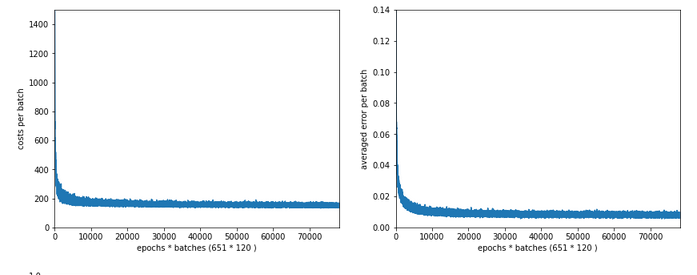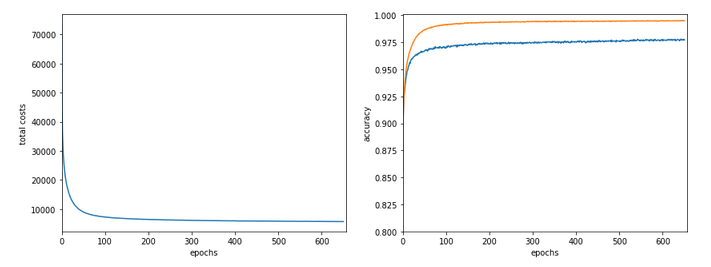```------------------
Starting epoch 651
....
....
avg total error of last mini_batch =  0.00878
presently reached train accuracy   =  0.99498
presently reached test accuracy    =  0.97740
presently batch averaged accuracy   =  0.99214
-------------------
Total training Time_CPU:  257.541123711002
```

The total time was to be expected as we checked accuracy values at each and every epoch both for the complete training and the test datasets (635/35*14 = 260 secs = 2.3 min!).

# Conclusion

This was a funny ride today. We found a major
impediment for a fast FW-propagation. We determined its cause in the inefficient combination of two differently ordered matrices which we used to account for bias nodes in the input layer. We investigated some optimization options for our present approach regarding bias neurons at layer L0. But it was much more reasonable to circumvent the whole problem by adding bias values already to the input array itself. This gave us a significant improvement for the FW-propagation of big batches – roughly by a factor of 2.5 for the complete training data set as an extreme example. But also testing accuracy on the full test data set at each and every epoch is no major performance factor any longer.

However, our whole analysis showed that we must put a big question mark behind our present approach to bias neurons. But before we attack this problem, we shall take a closer look at BW-propagation in the next article:

And there we shall replace another stupid time wasting part of the code, too. It will give us another improvement of around 15% to 20%. Stay tuned …

Performance of class methods vs. pure Python functions
stackoverflow : how-much-slower-python-classes-are-compared-to-their-equivalent-functions

Shuffle columns?
stackoverflow: shuffle-columns-of-an-array-with-numpy

Numpy arrays or matrices?
stackoverflow : numpy-np-array-versus-np-matrix-performance

# A simple Python program for an ANN to cover the MNIST dataset – XIV – cluster detection in feature space

We extend our studies of a program for a Multilayer perceptron and gradient descent in combination with the MNIST dataset:

In this article we shall work a bit on the following topic: How can we reduce the computational time required for gradient descent runs of our MLP?

Readers who followed my last articles will have noticed that I sometimes used 1800 epochs in a gradient descent run. The computational time including

• costly intermediate print outs into Jupyter cells,
• a full determination of the reached accuracy both on the full training and the test dataset at every epoch

lay in a region of 40 to 45 minutes for our MLP with two hidden layers and roughly 58000 weights. Using an Intel I7 standard CPU with OpenBlas
support. And I plan to work with bigger MLPs – not on MNIST but other data sets. Believe me: Everything beyond 10 minutes is a burden. So, I have a natural interest in accelerating things on a very basic level already before turning to GPUs or arrays of them.

# Factors for CPU-time

This introductory question leads to another one: What basic factors beyond technical capabilities of our Linux system and badly written parts of my Python code influence the consumption of computational time? Four points come to my mind; you probably find even more:

• One factor is certainly the extra forward propagation run which we apply to all samples of both the test and training data seat the end of each epoch. We perform this propagation to make predictions and to get data on the evolution of the accuracy, the total loss and the ratio of the regularization term to the real costs. We could do this in the future at every 2nd or 5th epoch to save some time. But this will reduce CPU-time only by less than 22%. 76% of the CPU-time of an epoch is spent in batch-handling with a dominant part in error backward propagation and weight corrections.
• The learning rate has a direct impact on the number of required epochs. We could enlarge the learning rate in combination with input data normalization; see the last article. This could reduce the number of required epochs significantly. Depending on the parameter choices before by up to 40% or 50%. But it requires a bit of experimenting ….
• Two other, more important factors are the frequent number of matrix operations during error back-propagation and the size of the involved matrices. These operations depend directly on the number of nodes involved. We could therefore reduce the number of nodes of our MLP to a minimum compatible with the required accuracy and precision. This leads directly to the next point.
• The dominant weight matrix is of course the one which couples layer L0 and layer L1. In our case its shape is 784 x 70; it has almost 55000 elements. The matrix for the next pair of layers has only 70×30 = 2100 elements – it is much, much smaller. To reduce CPU time for forward propagation we should try to make this matrix smaller. During error back propagation we must perform multiple matrix multiplications; the matrix dimensions depend on the number of samples in a mini-batch AND on the number of nodes in the involved layers. The dimensions of the the result matrix correspond to the those of the weight matrix. So once again: A reduction of the nodes in the first 2 layers would be extremely helpful for the expensive backward propagation. See: The math behind EBP.

# Reduction of the dimensions of the dominant matrix“requires a reduction of input features

The following numbers show typical CPU times spend for matrix operations during error back propagation [EBP] between different layers of our MLP and for two different batches at the beginning of gradient descent:

```Time_CPU for BW layer operations (to L2) 0.00029015699965384556
Time_CPU for BW layer operations (to L1) 0.0008645610000712622
Time_CPU for BW layer operations (to L0) 0.006551215999934357

Time_CPU for BW layer operations (to L2) 0.00029157400012991275
Time_CPU for BW layer operations (to L1) 0.0009575330000188842
Time_CPU for BW layer operations (to L0) 0.007488838999961445
```

The operations involving layer L0 cost a factor of 7 more CPU time than the other operations! Therefore, a key to the reduction of the number of mathematical operations is obviously the reduction of the number of nodes in the input layer! We cannot reduce the numbers in the hidden layers much, if we
do not want to hamper the accuracy properties of our MLP too much. So the basic question is

Can we reduce the number of input nodes somehow?

Yes, maybe we can! Input nodes correspond to „features„. In case of the MNIST dataset the relevant features are given by the gray-values for the 784 pixels of each image. A first idea is that there are many pixels within each MNIST image which are probably not used at all for classification – especially pixels at the outer image borders. So, it would be helpful to chop them off or to ignore them by some appropriate method. In addition, special significant pixel areas may exist to which the MLP, i.e. its weight optimization, reacts during training. For example: The digits 3, 5, 6, 8, 9 all have a bow within the lower 30% of an image, but in other regions, e.g. to the left and the right, they are rather different.

If we could identify suitable image areas in which dark pixels have a higher probability for certain digits then, maybe, we could use this information to discriminate the represented digits? But a „higher density of dark pixels in an image area“ is nothing else than a description of a „cluster“ of (dark) pixels in certain image areas. Can we use pixel clusters at numerous areas of an image to learn about the represented digits? Is the combination of (averaged) feature values in certain clusters of pixels representative for a handwritten digit in the MNIST dataset?

If the number of such pixel clusters could be reduced below lets say 100 then we could indeed reduce the number of input features significantly!

# Cluster detection

To be able to use relevant „clusters“ of pixels – if they exist in a usable form in MNIST images at all – we must first identify them. Cluster identification and discrimination is a major discipline of Machine Learning. This discipline works in general with unlabeled data. In the MNIST case we would not use the labels in the „y“-data at all to identify clusters; we would only use the „X“-data. A nice introduction to the mechanisms of cluster identification is given in the book of Paul Wilcott (see Machine Learning – book recommendations for the reference). The most fundamental method – called „kmeans“ – iterates over 3 major steps [I simplify a bit :-)]:

• We assume that K clusters exist and start with random initial positions of their centers (called „centroids“) in the multidimensional feature space
• We measure the distance of all data points to he centroids and associate a point with that centroid to which the distance is smallest
• We determine the „center of mass“ (according to some distance metric) of the identified data point groups and assume it as a new position of the centroids and move the old positions (a bit) in this direction.

We iterate over these steps until the centroids‘ positions hopefully get stable. Pretty simple. But there is a major drawback: You must make an assumption on the number „K“ of clusters. To make such an assumption can become difficult in the complex case of a feature space with hundreds of dimensions.

You can compensate this by executing multiple cluster runs and comparing the results. By what? Regarding the closure or separation of clusters in terms of an appropriate norm. One such norm is called „cluster inertia„; it measures the mean squared distance to the center for all points of a cluster. The theory is that the sum of the inertias for all clusters drops significantly with the number of clusters until an optimal number is reached and the inertia curve flattens out. The point where this happens in a plot of inertia vs. number of clusters is called „elbow„.
Identifying this „elbow“ is one of the means to find an optimal number of clusters. However, this recipe does not work under all circumstances. As the number of clusters get big we may be confronted with a smooth decline of the inertia sum.

# What data do we use for gradient descent after cluster detection?

How could we measure whether an image shows certain clusters? We could e.g. measure distances (with some appropriate metric) of all image points to the clusters. The „fit_transform()“-method of KMeans and MiniBatchKMeans provide us with with some distance measure of each image to the identified clusters. This means our images are transformed into a new feature space – namely into a „cluster-distance space“. This is a quite complex space, too. But it has less dimensions than the original feature space!

Note: We would of course normalize the resulting distance data in the new feature space before applying gradient descent.

# Application of „KMeansBatch“ to MNIST

There are multiple variants of „KMeans“. We shall use one which is provided by SciKit-Learn and which is optimized for large datasets: „MiniBatchKMeans„. It operates batch-wise without loosing too much of accuracy and convergence properties in comparison to KMeans (or a comparison see here). „MiniBatchKMeans“has some parameters you can play with.

We could be tempted to use 10 clusters as there are 10 digits to discriminate between. But remember: A digit can be written in very many ways. So, it is much more probable that we need a significant larger number of clusters. But again: How to determine on which K-values we should invest a bit more time? „Kmeans“ and methods alike offer another quantity called „silhouette“ coefficient. It measures how well the data points are within, at or outside the borders of a cluster. See the book of Geron referenced at the link given above on more information.

# Variation of CPU time, inertia and average silhouette coefficients with the number of clusters „K“

Let us first have a look at the evolution of CPU time, total inertia and averaged silhouette with the number of clusters „K“ for two different runs. The following code for a Jupyter cell gives us the data:

```
# *********************************************************
# Pre-Clustering => Searching for the elbow
# *********************************************************
from sklearn.cluster import KMeans
from sklearn.cluster import MiniBatchKMeans
from sklearn.preprocessing import StandardScaler
from sklearn.metrics import silhouette_score
X = np.concatenate((ANN._X_train, ANN._X_test), axis=0)
y = np.concatenate((ANN._y_train, ANN._y_test), axis=0)
print("X-shape = ", X.shape, "y-shape = ", y.shape)
num = X.shape

li_n = []
li_inertia = []
li_CPU = []
li_sil1 = []

# Loop over the number "n" of assumed clusters
rg_n = range(10,171,10)
for n in rg_n:
print("\nNumber of clusters: ", n)
start = time.perf_counter()
kmeans = MiniBatchKMeans(n_clusters=n, n_init=500, max_iter=1000, batch_size=500 )
X_clustered = kmeans.fit_transform(X)
sil1 = silhouette_score(X, kmeans.labels_)
#sil2 = silhouette_score(X_clustered, kmeans.labels_)
end = time.perf_counter()
dtime = end - start
print('Inertia = ', kmeans.inertia_)
print('Time_CPU = ', dtime)
print('sil1 score = ', sil1)
li_n.append(n)
li_inertia.append(kmeans.inertia_)
li_CPU.append(dtime)
li_sil1.append(sil1)

# Plots
# ******
fig_size = plt.rcParams["figure.figsize"]
fig_size[
0] = 14
fig_size = 5
fig1 = plt.figure(1)
fig2 = plt.figure(2)

ax1_1.plot(li_n, li_CPU)
ax1_1.set_xlabel("num clusters K")
ax1_1.set_ylabel("CPU time")

ax1_2.plot(li_n, li_inertia)
ax1_2.set_xlabel("num clusters K")
ax1_2.set_ylabel("inertia")

ax2_1.plot(li_n, li_sil1)
ax2_1.set_xlabel("num clusters K")
ax2_1.set_ylabel("silhoutte 1")

```

You see that I allowed for large numbers of initial centroid positions and iterations to be on the safe side. Before you try it yourself: Such runs for a broad variation of K-values are relatively costly. The CPU time rises from around 32 seconds for 30 clusters to a little less than 1 minute for 180 clusters. These times add up to a significant sum after a while …

Here are some plots: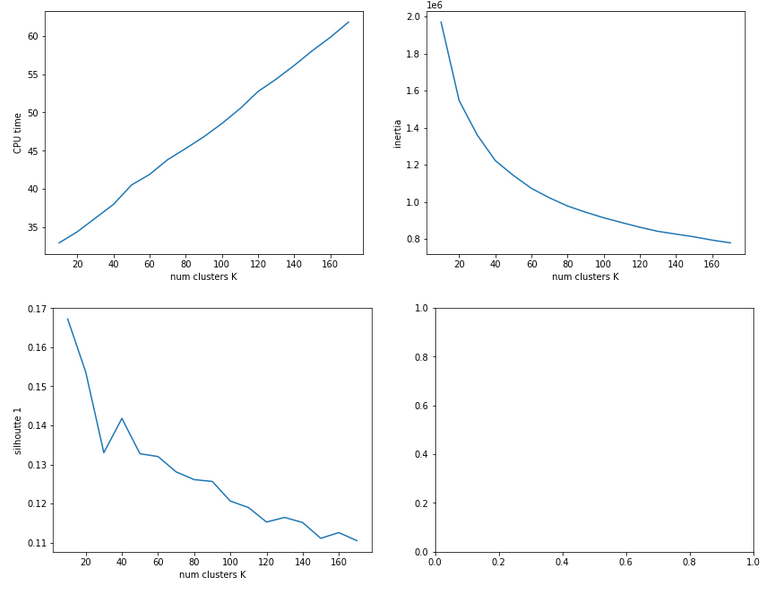The second run was executed with a higher resolution of K_(n+1) – K_n 5 = 5.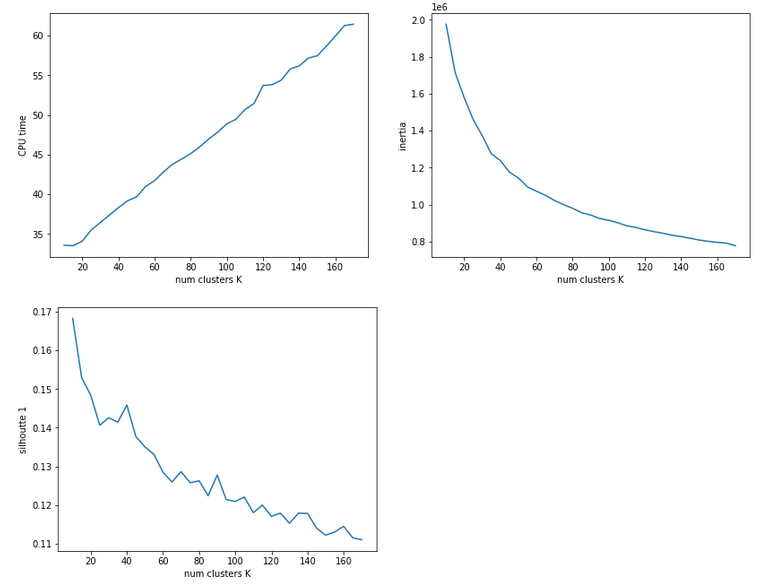We see that the CPU time to determine the centroids‘ positions varies fairly linear with „K“. And even for 170 clusters it does not take more than a minute! So, CPU-time for cluster identification is not a major limitation.

Unfortunately, we do not see a clear elbow in the inertia curve! What you regard as a reasonable choice for the number K depends a lot on where you say the curve starts to flatten. You could say that this happens around K = 60 to 90. But the results for the silhouette-quantity indicate for our parameter setting that K=40, K=70, K=90 are interesting points. We shall look at these points a bit closer with higher resolution later on.

# Reduction of the regularization factor (for Ridge regularization)

Now, I want to discuss an important point which I did not find in the literature:
In my last article we saw that regularization plays a significant but also delicate role in reaching top accuracy values for the test dataset. We saw that Lambda2 = 0.2 was a good choice for a normalized input of the MNIST data. It corresponded to a certain ratio of the regularization term to average batch costs.
But when we reduce the number of input nodes we also reduce the number of total weights. So the weight values themselves will automatically become bigger if we want to get to similar good values at the second layer. But as the regularization term depends in a quadratic way on the weights we may assume that we roughly need a linear reduction of Lambda2. So, for K=100 clusters we may shrink Lambda2 to (0.2/784*100) = 0.025 instead of 0.2. In general:

Lambda2_cluster = Lambda2_std * K / (number of input nodes)

I applied this rule of a thumb successfully throughout experiments with clustering befor gradient descent.

# Reference run without clustering

We saw at the end of article XII that we could reach an accuracy of around 0.975 after 500 epochs under optimal circumstances. But in the case I presented ten I was extremely lucky with the statistical initial weight distribution and the batch composition. In other runs with the same parameter setup I got smaller accuracy values. So, let us take an ad hoc run with the following parameters and results:
Parameters: learn_rate = 0.001, decrease_rate = 0.00001, mom_rate = 0.00005, n_size_mini_batch = 500, n_epochs = 600, Lambda2 = 0.2, weights at
all layers in [-2*1.0/sqrt(num_nodes_layer), 2*1.0/sqrt(num_nodes_layer)]
Results: acc_train: 0.9949 , acc_test: 0.9735, convergence after ca. 550-600 epochs

The next plot shows (from left to right and the down) the evolution of the costs per batch, the averaged error of the last mini-batch during an epoch, the ratio of regularization to batch costs and the total costs of the training set, respectively .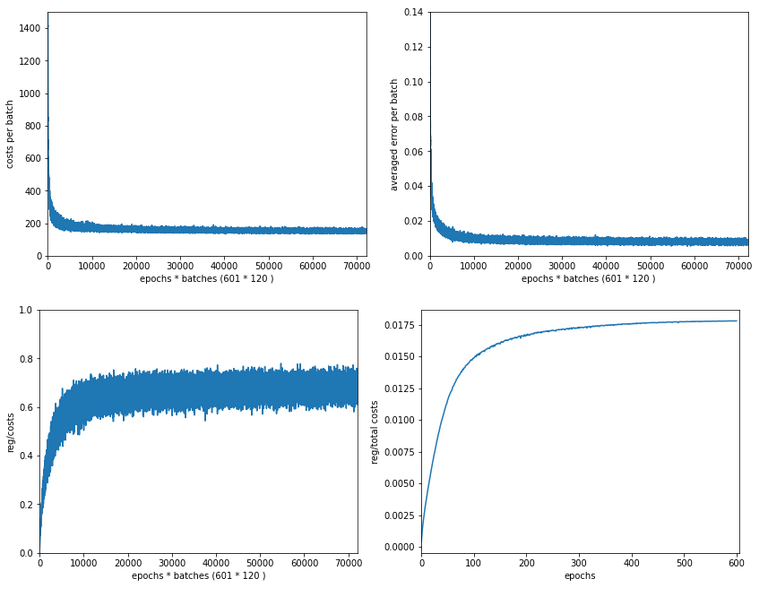The following plot summarizes the evolution of the total costs of the traaining set (including the regularization contribution) and the evolution of the accuracy on the training and the test data sets (in orange and blue, respectively).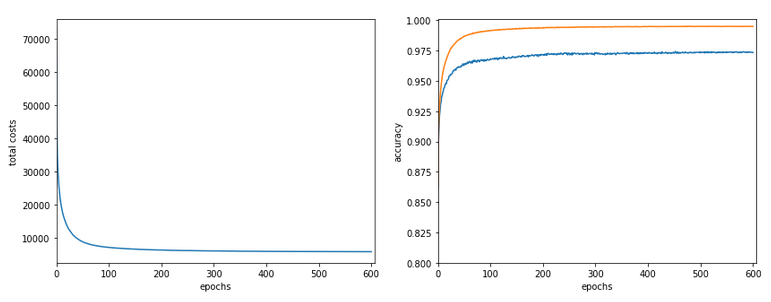The required computational time for the 600 epochs was roughly 18,2 minutes.

# Results of gradient descent based on a prior cluster identification

Before we go into a more detailed discussion of code adaption and test runs with things like clusters in unnormalized and normalized feature spaces, I want to show what we – without too much effort – can get out of using cluster detection ahead of gradient descent. The next plot shows the evolution of a run for K=70 clusters in combination with a special normalization: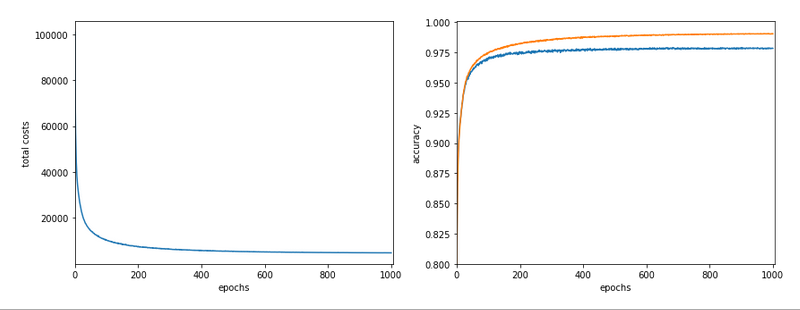and the total cost and accuracy evolution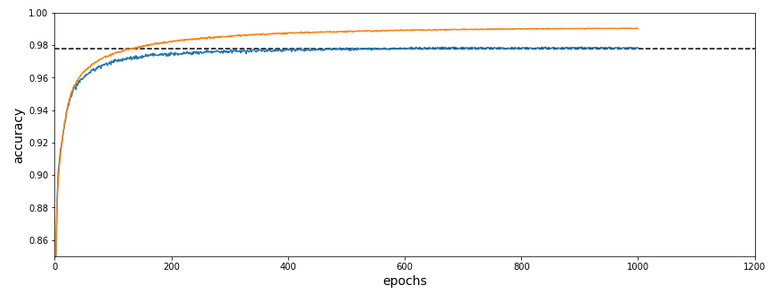The dotted line marks an accuracy of 97.8%! This is 0.5% bigger then our reference value of 97.3%. The total gain of %gt; 0.5% means however 18.5% of the remaining difference of 2.7% to 100% and we past a value of 97.8% already at epoch 600 of the run.

What were the required computational times?

If we just wanted 97.4% as accuracy we need around 150 epochs. And a total CPU time of 1.3 minutes to get to the same accuracy as our reference run. This is a factor of roughly 14 in required CPU time. For a stable 97.73% after epoch 350 we were still a factor of 5.6 better. For a stable accuracy beyond 97.8% we needed around 600 epochs – and still were by a factor of 3.3 faster than our reference run! So, clustering really brings some big advantages with it.

# Conclusion

In
this article I discussed the idea of introducing cluster identification in the (unnormalized or normalized) feature space ahead of gradient descent as a possible means to save computational time. A preliminary trial run showed that we indeed can become significantly faster by at least a factor of 3 up to 5 and even more. This is just due to the point that we reduced the number of input nodes and thus the number of mathematical calculations during matrix operations.

In the next article we shall have a more detailed look at clustering techniques in combination with normalization.

# A simple Python program for an ANN to cover the MNIST dataset – XII – accuracy evolution, learning rate, normalization

We continue our article series on building a Python program for a MLP and training it to recognize handwritten digits on images of the MNIST dataset.

In the last article we used our prediction data to build a so called „confusion matrix“ after training. With its help we got an overview about the „false negative“ and „false positive“ cases, i.e. cases of digit-images for which the algorithm made wrong predictions. We also displayed related critical MNIST images for the digit „4“.

In this article we first want to extend the ability of our class „ANN“ such that we can measure the level of accuracy (more precisely: the recall) on the full test and the training data sets during training. We shall see that the resulting curves will trigger some new insights. We shall e.g. get an answer to the question at which epoch the accuracy on the test data set does no longer change, but the accuracy on the training set still improves. Meaning: We can find out after which epoch we spend CPU time on overfitting.

In addition we want to investigate the efficiency of our present approach a bit. So far we have used a relatively small learning rate of 0.0001 with a decrease rate of 0.000001. This gave us relatively smooth curves during convergence. However, it took us a lot of epochs and thus computational time to arrive at a cost
minimum. The question is:

Is a small learning rate really required? What happens if we use bigger initial learning rates? Can we reduce the number of epochs until learning converges?

Regarding the last point we should not forget that a bigger learning rate may help to move out of local minima on our way to the vicinity of a global minimum. Some of our experiments will indeed indicate that one may get stuck somewhere before moving deep into a minimum valley. However, our forthcoming experiments will also show that we have to take care about the weight initialization. And this in turn will lead us to a major deficit of our present code. Resolving it will help us with bigger learning rates, too.

# Class interface changes

We introduce some new parameters, whose usage will become clear later on. They are briefly documented within the code. In addition we do no longer call the method _fit() automatically when we create a Python object instance of the class. This means the you have to call „_fit()“ on your own in your Jupyter cells in the future.

To be able to use some additional features later on we first need some more import statements.

New import statements of the class MyANN

```import numpy as np
import math
import sys
import time
import tensorflow
# from sklearn.datasets import fetch_mldata
from sklearn.datasets import fetch_openml
from sklearn.metrics import confusion_matrix
from sklearn.preprocessing import StandardScaler

from sklearn.cluster import KMeans
from sklearn.cluster import MiniBatchKMeans

from keras.datasets import mnist as kmnist
from scipy.special import expit
from matplotlib import pyplot as plt
from symbol import except_clause
from IPython.core.tests.simpleerr import sysexit
from math import floor
```

Extended My_ANN interface
We extend our interface substantially – although we shall not use every new parameter, yet. Most of the parameters are documented shortly; but to really understand what they control you have to look into some other changed parts of the class’s code, which we present later on. You can, however, safely ignore parameters on „clustering“ and „PCA“ in this article. We shall yet neither use the option to import MNIST X- and y-data (X_import, y_import) instead of loading them internally.

```
def __init__(self,
my_data_set = "mnist",

X_import = None, # imported X dataset
y_import = None, # imported y dataset

num_test_records = 10000, # number of test data

# parameter for normalization of input data
b_normalize_X = False, # True: apply StandardScaler on X input data

# parameters for clustering of input data
b_perform_clustering = False,  # shall we cluster the X_data before learning?
my_clustering_method = "MiniBatchKMeans", # Choice between 2 methods: MiniBatchKMeans, KMeans
cl_n_clusters = 200,      # number of clusters (often "k" in literature)
cl_max_iter = 600,        # number of iterations for centroid movement
cl_n_init = 100,          # number of different initial centroid positions tried
cl_n_jobs = 4,            # number of CPU cores (jobs to start for investigating n_init variations
cl_batch_size = 500,      # batch size, only used for MiniBatchKMeans

#parameters for PCA of input data
b_
perform_pca = False,
num_pca_categories = 155,

# parameters for MLP structure
n_hidden_layers = 1,
ay_nodes_layers = [0, 100, 0], # array which should have as much elements as n_hidden + 2
n_nodes_layer_out = 10,  # expected number of nodes in output layer

my_activation_function = "sigmoid",
my_out_function        = "sigmoid",
my_loss_function       = "LogLoss",

n_size_mini_batch = 50,  # number of data elements in a mini-batch

n_epochs      = 1,
n_max_batches = -1,  # number of mini-batches to use during epochs - > 0 only for testing
# a negative value uses all mini-batches

lambda2_reg = 0.1,     # factor for quadratic regularization term
lambda1_reg = 0.0,     # factor for linear regularization term

vect_mode = 'cols',

init_weight_meth_L0 = "sqrt_nodes",  # method to init weights => "sqrt_nodes", "const"
init_weight_meth_Ln = "sqrt_nodes",  # sqrt_nodes", "const"
init_weight_intervals = [(-0.5, 0.5), (-0.5, 0.5), (-0.5, 0.5)],   # size must fit number of hidden layers
init_weight_fact = 2.0,                # extends the interval

learn_rate = 0.001,          # the learning rate (often called epsilon in textbooks)
decrease_const = 0.00001,    # a factor for decreasing the learning rate with epochs
learn_rate_limit = 2.0e-05,  # a lower limit for the learn rate
reduction_fact = 0.001,      # small reduction factor - should be around 0.001 because of an exponential reduction

mom_rate   = 0.0005,         # a factor for momentum learning

b_shuffle_batches = True,    # True: we mix the data for mini-batches in the X-train set at the start of each epoch

b_predictions_train = False, # True: At the end of periodic epochs the code performs predictions on the train data set
b_predictions_test  = False, # True: At the end of periodic epochs the code performs predictions on the test data set
prediction_test_period  = 1, # Period of epochs for which we perform predictions
prediction_train_period = 1, # Period of epochs for which we perform predictions

print_period = 20,         # number of epochs for which to print the costs and the averaged error

figs_x1=12.0, figs_x2=8.0,
legend_loc='upper right',

b_print_test_data = True

):
'''
Initialization of MyANN
Input:
data_set: type of dataset; so far only the "mnist", "mnist_784" datsets are known
We use this information to prepare the input data and learn about the feature dimension.
This info is used in preparing the size of the input layer.

X_import: external X dataset to import
y_import: external y dataset to import - must fit in dimension to X_import

num_test_records: number of test data

b_normalize_X: True => Invoke the StandardScaler of
Scikit-Learn
to center and normalize the input data X

Preprocessing of input data treatment before learning
------------------------------------
Clustering
-----------
b_perform_clustering   # True => Cluster the X_data before learning?
my_clustering_method   # string: 2 methods: MiniBatchKMeans, KMeans
cl_n_clusters = 200       # number of clusters (often "k" in literature)
cl_max_iter = 600      # number of iterations for centroid movement
cl_n_init = 100        # number of different initial centroid positions tried
cl_n_jobs = 4,         # number of CPU cores => jobs - only used for "KMeans"
cl_batch_size = 500    # batch size used for "MiniBatchKMeans"

PCA
-----
b_perform_pca: True => perform a pca analysis
num_pca_categories: 155 - choose a reasonable number

n_hidden_layers = number of hidden layers => between input layer 0 and output layer n

ay_nodes_layers = [0, 100, 0 ] : We set the number of nodes in input layer_0 and the output_layer to zero
Will be set to real number afterwards by infos from the input dataset.
All other numbers are used for the node numbers of the hidden layers.
n_nodes_out_layer = expected number of nodes in the output layer (is checked);
this number corresponds to the number of categories NC = number of labels to be distinguished

my_activation_function : name of the activation function to use
my_out_function : name of the "activation" function of the last layer which produces the output values
my_loss_function : name of the "cost" or "loss" function used for optimization

n_size_mini_batch : Number of elements/samples in a mini-batch of training data
The number of mini-batches will be calculated from this

n_epochs : number of epochs to calculate during training
n_max_batches : > 0: maximum of mini-batches to use during training
< 0: use all mini-batches

lambda_reg2:    The factor for the quadartic regularization term
lambda_reg1:    The factor for the linear regularization term

vect_mode: Are 1-dim data arrays (vctors) ordered by columns or rows ?

init_weight_meth_L0: Method to calculate the initial weights at layer L0: "sqrt_nodes" => sqrt(number of nodes) /  "const" => interval borders
init_weight_meth_Ln: Method to calculate the initial weights at hidden layers
init_weight_intervals: list of tuples with interval limits [(-0.5, 0.5), (-0.5, 0.5), (-0.5, 0.5)],
size must fit number of hidden layers
init_weight_fact:  interval limits get scald by this factor, e.g. 2* (0,5, 0.5)

learn rate :     Learning rate - definies by how much we correct weights in the indicated direction of the gradient on the cost hyperplane.
decrease_const:  Controls a systematic decrease of the learning rate with epoch number
learn_rate_limit = 2.0e-05,  # a lowee limit for the learning rate

reduction_fact:  around 0.001  => almost exponential reduction during the first 500 epochs

mom_const:       Momentum rate. Controls a mixture of the last with the present weight
corrections (momentum learning)

b_shuffle_batches: True => vary composition of mini-batches with each epoch

# The next two parameters enable the measurement of accuracy and total cost function
# by making predictions on the train and test datasets
b_predictions_train: True => perform a prediction run on the full training data set => get accuracy
b_predictions_test:  True => perform a prediction run on the full test data set => get accuracy
prediction_test_period: period of epochs for which to perform predictions
prediction_train_period: period of epochs for which to perform predictions

print_period:    number of periods between printing out some intermediate data
on costs and the averaged error of the last mini-batch

figs_x1=12.0, figs_x2=8.0 : Standard sizing of plots ,
legend_loc='upper right': Position of legends in the plots

b_print_test_data: Boolean variable to control the print out of some tests data

'''

# Array (Python list) of known input data sets
self._input_data_sets = ["mnist", "mnist_784", "mnist_keras", "imported"]
self._my_data_set = my_data_set

# X_import, y_import, X, y, X_train, y_train, X_test, y_test
# will be set by method handle_input_data()
# X: Input array (2D) - at present status of MNIST image data, only.
# y: result (=classification data) [digits represent categories in the case of Mnist]
self._X_import = X_import
self._y_import = y_import

# number of test data
self._num_test_records = num_test_records

self._X       = None
self._y       = None
self._X_train = None
self._y_train = None
self._X_test  = None
self._y_test  = None

# perform a normalization of the input data
self._b_normalize_X = b_normalize_X

# relevant dimensions
# from input data information;  will be set in handle_input_data()
self._dim_X        = 0  # total num of records in the X,y input sets
self._dim_sets     = 0  # num of records in the TRAINING sets X_train, y_train
self._dim_features = 0
self._n_labels     = 0   # number of unique labels - will be extracted from y-data

# Img sizes
self._dim_img      = 0 # should be sqrt(dim_features) - we assume square like images
self._img_h        = 0
self._img_w        = 0

# Preprocessing of input data
# ---------------------------
self._b_perform_clustering = b_perform_clustering
self._my_clustering_method = my_clustering_method # for the related dictionary see below
self._kmeans        = None   # pointer to object used for clustering
self._cl_n_clusters = cl_n_clusters       # number of clusters (often "k" in literature)
self._cl_max_iter   = cl_max_iter      # number of iterations for centroid movement
self._cl_n_init     = cl_n_init        # number of different initial centroid positions tried
self._cl_batch_size = cl_batch_size    # batch size used for MiniBatchKMeans
self._cl_n_jobs     = cl_n_jobs        # number of parallel jobs (on CPU-cores) - only used for KMeans

# Layers
# ------
# number of hidden layers
self._n_hidden_layers = n_hidden_layers
# Number of total layers
self._n_total_layers = 2 + self._n_hidden_layers
# Nodes for hidden layers

self._ay_nodes_layers = np.array(ay_nodes_layers)
# Number of nodes in output layer - will be checked against information from target arrays
self._n_nodes_layer_out = n_nodes_layer_out

# Weights
# --------
# empty List for all weight-matrices for all layer-connections
# Numbering :
# w contains the weight matrix which connects layer 0 (input layer ) to hidden layer 1
# w contains the weight matrix which connects layer 1 (input layer ) to (hidden?) layer 2
self._li_w = []

# Arrays for encoded output labels - will be set in _encode_all_mnist_labels()
# -------------------------------
self._ay_onehot = None
self._ay_oneval = None

# Known Randomizer methods ( 0: np.random.randint, 1: np.random.uniform )
# ------------------
self.__ay_known_randomizers = [0, 1]

# Types of activation functions and output functions
# ------------------
self.__ay_activation_functions = ["sigmoid"] # later also relu
self.__ay_output_functions     = ["sigmoid"] # later also softmax

# Types of cost functions
# ------------------
self.__ay_loss_functions = ["LogLoss", "MSE" ] # later also other types of cost/loss functions

# dictionaries for indirect function calls
self.__d_activation_funcs = {
'sigmoid': self._sigmoid,
'relu':    self._relu
}
self.__d_output_funcs = {
'sigmoid': self._sigmoid,
'softmax': self._softmax
}
self.__d_loss_funcs = {
'LogLoss': self._loss_LogLoss,
'MSE': self._loss_MSE
}
# Derivative functions
self.__d_D_activation_funcs = {
'sigmoid': self._D_sigmoid,
'relu':    self._D_relu
}
self.__d_D_output_funcs = {
'sigmoid': self._D_sigmoid,
'softmax': self._D_softmax
}
self.__d_D_loss_funcs = {
'LogLoss': self._D_loss_LogLoss,
'MSE': self._D_loss_MSE
}
self.__d_clustering_functions = {
'MiniBatchKMeans': self._Mini_Batch_KMeans,
'KMeans': self._KMeans
}

# The following variables will later be set by _check_and set_activation_and_out_functions()
self._my_act_func  = my_activation_function
self._my_out_func  = my_out_function
self._my_loss_func = my_loss_function
self._act_func      = None
self._out_func      = None
self._loss_func     = None
self._cluster_func  = None

# number of data samples in a mini-batch
self._n_size_mini_batch = n_size_mini_batch
self._n_mini_batches = None  # will be determined by _get_number_of_mini_batches()

# maximum number of epochs - we set this number to an assumed maximum
# - as we shall build a backup and reload functionality for training, this should not be a major problem
self._n_epochs = n_epochs

# maximum number of batches to handle ( if < 0 => all!)
self._n_max_batches = n_max_batches
# actual number of batches
self._n_batches = None

# regularization parameters
self._lambda2_reg = lambda2_reg
self._lambda1_reg = lambda1_reg

# parameters to control the initialization of the weights (see _create_WM_Input(), create_WM_Hidden())
self._init_weight_meth_L0 = init_weight_meth_L0
self._init_weight_meth_Ln = init_weight_meth_Ln
self._init_weight_
intervals = init_weight_intervals # list of lists with interval borders
self._init_weight_fact = init_weight_fact           # extends weight intervals

# parameters for adaption of the learning rate
self._learn_rate = learn_rate
self._decrease_const = decrease_const
self._learn_rate_limit = learn_rate_limit
self._reduction_fact  = reduction_fact
#
# parameters for momentum learning
self._mom_rate   = mom_rate
self._li_mom = [None] *  self._n_total_layers

# shuffle data in X_train?
self._b_shuffle_batches = b_shuffle_batches

# perform predictions on train and test data set and related analysis
self._b_predictions_train = b_predictions_train
self._b_predictions_test  = b_predictions_test
self._prediction_test_period  = prediction_test_period
self._prediction_train_period = prediction_train_period

# epoch period for printing
self._print_period = print_period

# book-keeping for epochs and mini-batches
# -------------------------------
# range for epochs - will be set by _prepare-epochs_and_batches()
self._rg_idx_epochs = None
# range for mini-batches
self._rg_idx_batches = None
# dimension of the numpy arrays for book-keeping - will be set in _prepare_epochs_and_batches()
self._shape_epochs_batches = None    # (n_epochs, n_batches, 1)

# training evolution:
# +++++++++++++++++++
# List for error values at outermost layer for minibatches and epochs during training
# we use a numpy array here because we can redimension it
self._ay_theta = None
# List for cost values of mini-batches during training - The list will later be split into sections for epochs
self._ay_costs = None
#
# List for test accuracy values and error values at epoch periods
self._ay_period_test_epoch = None # x-axis for plots of the following 2 quantities
self._ay_acc_test_epoch = None
self._ay_err_test_epoch = None
# List for train accuracy values and error values at epoch periods
self._ay_period_train_epoch = None # x-axis for plots of the following 2 quantities
self._ay_acc_train_epoch = None
self._ay_err_train_epoch = None

# Data elements for back propagation
# ----------------------------------

# 2-dim array of partial derivatives of the elements of an additive cost function
# The derivative is taken with respect to the output results a_j = ay_ANN_out[j]
# The array dimensions account for nodes and sampls of a mini_batch. The array will be set in function
# self._initiate_bw_propagation()
self._ay_delta_out_batch = None

# parameter to allow printing of some test data
self._b_print_test_data = b_print_test_data

# Plot handling
# --------------
# Alternatives to resize plots
# 1: just resize figure  2: resize plus create subplots() [figure + axes]
self._plot_resize_alternative = 1
# Plot-sizing
self._figs_x1 = figs_x1
self._figs_x2 = figs_x2
self._fig = None
self._ax  = None
# alternative 2 does resizing and (!) subplots()
self.initiate_and_resize_plot(self._plot_resize_alternative)

# ***********
# operations
# ***********

# check and handle input data
self._handle_input_data()
# set the ANN structure
self._set_ANN_structure()

# Prepare epoch and batch-handling - sets ranges, limits num of mini-batches and initializes book-keeping arrays
self._rg_idx_epochs, self._rg_idx_batches = self._prepare_epochs_and_batches()
```

# Code modifications to create precise accuracy information on the full test and training sets during training

You certainly noticed the following set of control parameters in the class’s new interface:

• b_predictions_train = False, # True: At the end of periodic epochs the code performs predictions on the train data set
• b_predictions_test = False, # True: At the end of periodic epochs the code performs predictions on the test data set
• prediction_test_period = 1, # Period of epochs for which we perform predictions
• prediction_train_period = 1, # Period of epochs for which we perform predictions

These parameters control whether we perform predictions during training for the full test dataset and/or the full training dataset – and if so, at which epoch period. Actually, during all of the following experiments we shall evaluate the accuracy data after each single period.

We need an array to gather accuracy information. We therefore modify the method „_prepare_epochs_and_batches()“, where we fill some additional Numpy arrays with initialization values. Thus we avoid a costly „append()“ later on; we just overwrite the array entries successively. This overwriting happens in our method _fit(); see below.

Changes to function „_prepare_epochs_and_batches()“:

```    ''' -- Main Method to prepare epochs, batches and book-keeping arrays -- '''
def _prepare_epochs_and_batches(self, b_print = True):
# range of epochs
ay_idx_epochs  = range(0, self._n_epochs)

# set number of mini-batches and array with indices of input data sets belonging to a batch
self._set_mini_batches()

# limit the number of mini-batches
self._n_batches = min(self._n_max_batches, self._n_mini_batches)
ay_idx_batches = range(0, self._n_batches)
if (b_print):
if  self._n_batches < self._n_mini_batches :
print("\nWARNING: The number of batches has been limited from " +
str(self._n_mini_batches) + " to " + str(self._n_max_batches) )

# Set the book-keeping arrays
self._shape_epochs_batches = (self._n_epochs, self._n_batches)
self._ay_theta = -1 * np.ones(self._shape_epochs_batches) # float64 numbers as default
self._ay_costs = -1 * np.ones(self._shape_epochs_batches) # float64 numbers as default

shape_test_epochs  = ( floor(self._n_epochs / self._prediction_test_period), )
shape_train_epochs = ( floor(self._n_epochs / self._prediction_train_period), )
self._ay_period_test_epoch  = -1 * np.ones(shape_test_epochs) # float64 numbers as default
self._ay_acc_test_epoch     = -1 * np.ones(shape_test_epochs) # float64 numbers as default
self._ay_err_test_epoch     = -1 * np.ones(shape_test_epochs) # float64 numbers as default
self._ay_period_train_epoch = -1 * np.ones(shape_train_epochs) # float64 numbers as default
self._ay_acc_train_epoch    = -1 * np.ones(shape_train_epochs) # float64 numbers as default
self._ay_err_train_epoch    = -1 * np.ones(shape_train_epochs) # float64 numbers as default

return ay_idx_epochs, ay_idx_batches
#
```

We then create two new methods to calculate accuracy values by predicting results on all records of both the training dataset and the test dataset. The attentive reader certainly recognizes the methods‘ structure from a previous article where we used
similar code in a Jupyter cell:

New functions „_predict_all_test_data()“ and „_predict_all_train_data()“:

```    ''' Method to predict values for the full set of test data '''
def _predict_all_test_data(self):
size_set = self._X_test.shape

li_Z_in_layer_test  = [None] * self._n_total_layers
li_Z_in_layer_test = self._X_test

# Transpose input data matrix
ay_Z_in_0T       = li_Z_in_layer_test.T
li_Z_in_layer_test = ay_Z_in_0T
li_A_out_layer_test  = [None] * self._n_total_layers

# prediction by forward propagation of the whole test set
self._fw_propagation(li_Z_in = li_Z_in_layer_test, li_A_out = li_A_out_layer_test, b_print = False)
ay_predictions_test = np.argmax(li_A_out_layer_test[self._n_total_layers-1], axis=0)

# accuracy
ay_errors_test = self._y_test - ay_predictions_test
acc_test = (np.sum(ay_errors_test == 0)) / size_set
# print ("total acc for test data = ", acc)
# return acc, ay_predictions_test
return acc_test
#
''' Method to predict values for the full set of training data '''
def _predict_all_train_data(self):
size_set = self._X_train.shape

li_Z_in_layer_train  = [None] * self._n_total_layers
li_Z_in_layer_train = self._X_train
# Transpose
ay_Z_in_0T       = li_Z_in_layer_train.T
li_Z_in_layer_train = ay_Z_in_0T
li_A_out_layer_train  = [None] * self._n_total_layers

self._fw_propagation(li_Z_in = li_Z_in_layer_train, li_A_out = li_A_out_layer_train, b_print = False)
ay_predictions_train = np.argmax(li_A_out_layer_train[self._n_total_layers-1], axis=0)
ay_errors_train = self._y_train - ay_predictions_train
acc_train = (np.sum(ay_errors_train == 0)) / size_set
#print ("total acc for train data = ", acc)

return acc_train
#
```

Eventually, we modify our method „_fit()“ with a series of statements on the level of the epoch loop. You may ignore most of the statements for learning rate adaption; we only use the „simple“ adaption methods. The really important changes are those regarding predictions.

Modifications of function „_fit()“:

```    ''' -- Method to perform training in epochs for defined mini-batches -- '''
def _fit(self, b_print = False, b_measure_epoch_time = True, b_measure_batch_time = False):
'''
Parameters:
b_print:                 Do we print intermediate results of the training at all?
b_print_period:          For which period of epochs do we print?
b_measure_epoch_time:    Measure CPU-Time for an epoch
b_measure_batch_time:    Measure CPU-Time for a batch
'''
rg_idx_epochs  = self._rg_idx_epochs
rg_idx_batches = self._rg_idx_batches
if (b_print):
print("\nnumber of epochs = " + str(len(rg_idx_epochs)))
print("max number of batches = " + str(len(rg_idx_batches)))

# Some intial parameters
acc_old = 0.0000001
acc_test = 0.001
orig_rate = self._learn_rate
n_predict_test  = 0
n_predict_train = 0

# loop over epochs
# ****************
start_train = time.perf_counter()
for idxe in rg_idx_epochs:
if b_print and (idxe % self._print_period == 0):
if b_measure_epoch_time:

start_0_e = time.perf_counter()
print("\n ---------")
print("Starting epoch " + str(idxe+1))

# simple adaption of the learning rate
# ~~~~~~~~~~~~~~~~~~~~~~~~~~~~~~~~~~~~
orig_rate /= (1.0 + self._decrease_const * idxe)
self._learn_rate /= (1.0 + self._decrease_const * idxe)
if self._learn_rate < self._learn_rate_limit:
self._learn_rate = self._learn_rate_limit

# adapt wit acc. - not working well, yet
#~~~~~~~~~~~~~~~~~~~~~~~~~~~~~~~~~~~~~~~
acc_change_rate = math.fabs((acc_test - acc_old) / acc_old)
if b_print and (idxe % self._print_period == 0):
print("acc_chg_rate = ", acc_change_rate)
ratio = self._learn_rate / orig_rate
if ratio > 0.33 and acc_change_rate < 1/3 and self._adapt_with_acc:
if acc_change_rate < 0.001:
acc_change_rate = 0.001
#adapt_fact = 2.0 * acc_change_rate / (1.0 - acc_change_rate)
adapt_fact = 1.0 - 0.001 * (1.0 - acc_change_rate / (1.0 - acc_change_rate))
if b_print and (idxe % self._print_period == 0):
acc_old = acc_test # for adaption of learning rate

# shuffle indices for a variation of the mini-batches with each epoch
# ******************************************************************
if self._b_shuffle_batches:
shuffled_index = np.random.permutation(self._dim_sets)
self._X_train, self._y_train, self._ay_onehot = self._X_train[shuffled_index], self._y_train[shuffled_index], self._ay_onehot[:, shuffled_index]
#
# loop over mini-batches
# **********************
for idxb in rg_idx_batches:
if b_measure_batch_time:
start_0_b = time.perf_counter()
# deal with a mini-batch
self._handle_mini_batch(num_batch = idxb, num_epoch=idxe, b_print_y_vals = False, b_print = False)
if b_measure_batch_time:
end_0_b = time.perf_counter()
print('Time_CPU for batch ' + str(idxb+1), end_0_b - start_0_b)

#
# predictions
# ***********
# Control and perform predictions on the full test data set
if self._b_predictions_test and idxe % self._prediction_test_period == 0:
self._ay_period_test_epoch[n_predict_test] = idxe
acc_test = self._predict_all_test_data()
self._ay_acc_test_epoch[n_predict_test] = acc_test
n_predict_test += 1
# Control and perform predictions on the full training training data set
if self._b_predictions_train and idxe % self._prediction_train_period == 0:
self._ay_period_train_epoch[n_predict_train] = idxe
acc_train = self._predict_all_train_data()
self._ay_acc_train_epoch[n_predict_train] = acc_train
n_predict_train += 1
#
# printing some evolution and epoch information
if b_print and (idxe % self._print_period == 0):
if b_measure_epoch_time:
end_0_e = time.perf_counter()
print('Time_CPU for epoch' + str(idxe+1), end_0_e - start_0_e)
print("learning rate = ", self._learn_rate)
print("orig learn rate = ", orig_rate)
print("\ntotal costs of last mini_batch = ", self._ay_costs[idxe, idxb])
print("avg total error of
last mini_batch = ", self._ay_theta[idxe, idxb])
# print presently reached accuracy values on the test and training sets
print("presently reached train accuracy =<div style="width: 95%; overflow: auto; height: 400px;">
<pre style="width: 1000px;">
", acc_train)
print("presently reached test accuracy = ", acc_test)

# print out required secs for training
# **************************************
end_train = time.perf_counter()
print('\n\n ------')
print('Total training Time_CPU: ', end_train - start_train)
print("\nStopping program regularily")

return None
#
```

The method we apply in the above code to reduce the learning rate with every epoch is by the way called „power scheduling„. The book of Aurelien Geron [„Hands on Machine learning ….“, 2019, 2nd edition, O’Reilly], quoted already in previous articles, lists a bunch of other methods, e.g. „exponential scheduling“, where we multiply the learning rate with a constant factor < 1 at every epoch.

A further change of code happens in the functions _create_WM_input() and _ceate_WM_hidden“ to initiate weight values.

Modifications of functions _create_WM_input() and _create_WM_hidden“:
Addendum 25.03.2020: Changed _create_WM_hidden() because of errors in the code

```    '''-- Method to create the weight matrix between L0/L1 --'''
def _create_WM_Input(self):
'''
Method to create the input layer
The dimension will be taken from the structure of the input data
We need to fill self._w with a matrix for conections of all nodes in L0 with all nodes in L1
We fill the matrix with random numbers between [-1, 1]
'''
# the num_nodes of layer 0 should already include the bias node
num_nodes_layer_0 = self._ay_nodes_layers
num_nodes_with_bias_layer_0 = num_nodes_layer_0 + 1
num_nodes_layer_1 = self._ay_nodes_layers

# Set interval borders for randomizer
if self._init_weight_meth_L0 == "sqrt_nodes":  # sqrtr(nodes) - rule of Prof. J. Frochte
rand_high = self._init_weight_fact / math.sqrt(float(num_nodes_layer_0))
rand_low = - rand_high
else:
rand_low  = self._init_weight_intervals
rand_high = self._init_weight_intervals
print("\nL0: weight range [" + str(rand_low) + ", " + str(rand_high) + "]" )

# fill the weight matrix at layer L0 with random values
randomizer = 1 # method np.random.uniform
rand_size = num_nodes_layer_1 * (num_nodes_with_bias_layer_0)
w0 = self._create_vector_with_random_values(rand_low, rand_high, rand_size, randomizer)
w0 = w0.reshape(num_nodes_layer_1, num_nodes_with_bias_layer_0)

# put the weight matrix into array of matrices
self._li_w.append(w0)
print("\nShape of weight matrix between layers 0 and 1 " + str(self._li_w.shape))

#
'''-- Method to create the weight-matrices for hidden layers--'''
def _create_WM_Hidden(self):
'''
Method to create the weights of the hidden layers, i.e. between [L1, L2] and so on ... [L_n, L_out]
We fill the matrix with random numbers between [-1, 1]
'''

# The "+1" is required due to range properties !
rg_hidden_layers = range(1, self._n_hidden_layers + 1, 1)

# Check parameter input fro weight intervals
if self._init_weight_meth_Ln == "const":
if len(self._init_
weight_intervals) != (self._n_hidden_layers + 1):
print("\nError: we shall initialize weights with values from intervals, but wrong number of intervals provided!")
sys.exit()

for i in rg_hidden_layers:
print ("\nCreating weight matrix for layer " + str(i) + " to layer " + str(i+1) )

num_nodes_layer = self._ay_nodes_layers[i]
num_nodes_with_bias_layer = num_nodes_layer + 1

# Set interval borders for randomizer
if self._init_weight_meth_Ln == "sqrt_nodes":  # sqrtr(nodes) - rule of Prof. J. Frochte
rand_high = self._init_weight_fact / math.sqrt(float(num_nodes_layer))
rand_low = - rand_high
else:
rand_low  = self._init_weight_intervals[i]
rand_high = self._init_weight_intervals[i]
print("L" + str(i) + ": weight range [" + str(rand_low) + ", " + str(rand_high) + "]" )

# the number of the next layer is taken without the bias node!
num_nodes_layer_next = self._ay_nodes_layers[i+1]

# ill the weight matrices at the hidden layer with random values
rand_size = num_nodes_layer_next * num_nodes_with_bias_layer
randomizer = 1 # np.random.uniform
w_i_next = self._create_vector_with_random_values(rand_low, rand_high, rand_size, randomizer)
w_i_next = w_i_next.reshape(num_nodes_layer_next, num_nodes_with_bias_layer)

# put the weight matrix into our array of matrices
self._li_w.append(w_i_next)
print("Shape of weight matrix between layers " + str(i) + " and " + str(i+1) + " = " + str(self._li_w[i].shape))
#
```

As you see, we distinguish between different cases depending on the parameters „init_weight_meth_L0“ and „init_weight_meth_Ln“. There, obviously, happens a choice regarding the borders of the intervals from which we randomly pick our initial weight values. In case of the method „sqrt_nodes“ the interval borders are determined by the number of nodes of the neighboring layer. Otherwise we can read the interval borders from the parameter list „init_weight_intervals“. You will better understand these options later on.

# Plotting accuracys

We use a very simple code in a Jupyter cell to get a plot for the accuracy values on the training and the test datasets. The orange line will show the accuracy reached at each epoch for the training dataset when we apply the weights evaluated given at the epoch. The blue line shows the accuracy reached for the test dataset. In the text below we shall use the following abbreviations:

acc_train = accuracy reached for the X_train dataset of MNIST
acc_test   = accuracy reached for the X_test dataset of MNIST

Code for plotting

```y_min=0.75
y_max=1.0
plt.xlim(0,1800)
plt.ylim(y_min, y_max)

xplot=ANN._ay_period_test_epoch
yplot=ANN._ay_acc_test_epoch
plt.plot(xplot,yplot)

xplot=ANN._ay_period_train_epoch
yplot=ANN._ay_acc_train_epoch
plt.plot(xplot,yplot)
plt.show()
```

# Experiment 1: Accuracy plot for a Reference Run

The next test run will be used as a reference run for comparisons later on. It shows where we stand right now.

Test 1:
Parameters: learn_rate = 0.0001, decrease_rate = 0.000001, mom_rate = 0.00005, n_size_mini_batch = 500, Lambda2 = 0.2, n_epochs = 1800, initial weights for all layers in [-0.5, +0.5]:
Results: acc_train: 0.996 , acc_test: 0.961, convergence after ca. 1150 epochs

Note that we use a very small learn_rate, but an even smaller decrease rate. The
evolution of the accuracy values looks like follows: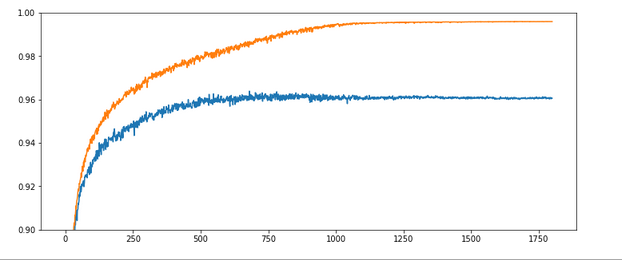The x-axis measures the number of epochs during training. The y-axis the degree of accuracy – given as a fraction; multiply by 100 to get percentage values. By the way, applying the reached weight set on the full training and test datasets in each epoch cost us at least 20% rise in CPU time (45 minutes).

What does our new way of representing the „learning“ of our MLP by the evolution of the accuracy levels tell us?

Noise: There is substantial noise visible along the lines. If you go back to previous articles you may detect the same type of noise in the plots of the evolution of the cost function. Obviously, our mini-batches and the constant change of their composition with each epoch lead to wiggles around the visible trends.

Tendencies: Note that there is a tendency of linear rise of the accuracy acc_train between periods 350 and 900. And, actually, the accuracy even decreases a bit around epoch 1550. This is a warning that the very last epoch of a run may not reveal the optimal solution.

Overfitting and a typical related splitting of the evolution of the two accuracys: One clearly sees that after a certain epoch (here: around epoch 300) the accuracy on the training dataset deviates systematically from the accuracy on the test dataset. In the end the gap is bigger than 3.5 percent. And in our case the accuracy on the test dataset reaches its final level of 0.96 significantly earlier – at around epoch 750 – and remains there, while the accuracy on the training set still rises up to epoch 1000.

However, I would like to add a warning:
Warning: Later on we shall see that there are cases for which both curves turn into a convergence at almost the same epoch. So, yes, there almost always occurs some overfitting during training of a MLP. However, we cannot set up a rule which says that convergence of the accuracy on the test dataset always occurs much earlier than for the training set. You always have to watch the evolution of both during your training experiments!

# Experiment 2: Increasing the learning rate – better efficiency?

Let us now be brave and increase the learning rate by a factor of 10:

Test 2:
Parameters: learn_rate = 0.001, decrease_rate = 0.000001, mom_rate = 0.00005, n_size_mini_batch = 500, Lambda2 = 0.2, n_epochs = 1800, initial weights for all layers in [-0.5, +0.5]:
Results: acc_train: 0.971 , acc_test: 0.959, no convergence after ca. 1800 epochs, yet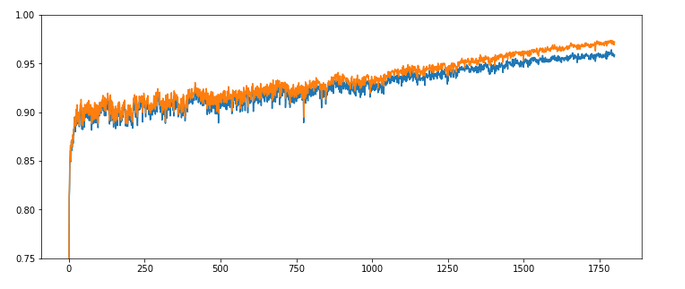Ooops! Our algorithm ran into real difficulties! We seem to hop in and out of a minimum area until epoch 400 and despite a following systematic linear improvementthere is no sign of a real convergence – yet!

The learning rate seems to big to lead to a consistent quick path into a minimum of all mini-batches! This may have to do with the size of the mini-batches, too – see below. The increase of the learning rate did not do us any good.

# Experiment 3: Increased learning rate – but a higher decrease rate, too

As the larger learning rate seems to be a problem after period 50, we may think of a faster reduction of the learning rate.

Test 3:
Parameters: learn_rate = 0.001, decrease_rate = 0.00001, mom_rate = 0.00005, n_
size_mini_batch = 500, Lambda2 = 0.2, n_epochs = 2000, initial weights for all layers in [-0.5, +0.5]:
Results: acc_train: 0.9909, acc_test: 0.9646, convergence after ca. 800 epochs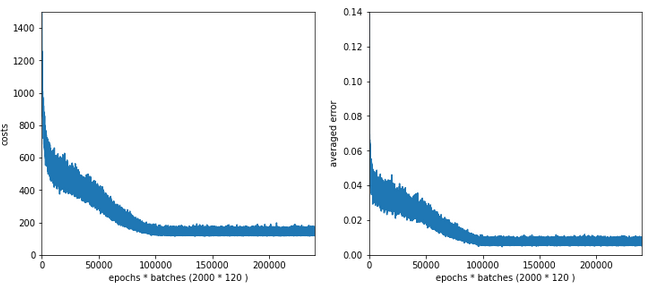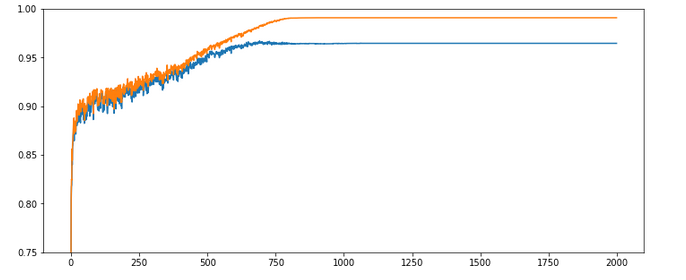The evolution looks strange, too, but better than experiment 2! We see a real convergence again after some rather linear development! As a lesson learned I would say: Yes we can work with an initially bigger learning rate – but we need a stronger decrease of it, too, to really run into a global minimum eventually.

# Experiment 4: Increased learning rate, higher decrease rate and smaller initial weights

Maybe the weight initialization has some impact? According to a rule published by Prof. Frochte in his book „Maschinelles Lernen“ [2019, 2. Auflage, Carl Hanser Verlag] I limited the initial random weight values to a range between [-1.0/sqrt(784), +1.0/sqrt(784)] – instead of [-0.5, 0.5] for all layers.

Test 4:
Parameters: learn_rate = 0.001, decrease_rate = 0.00001, mom_rate = 0.00005, n_size_mini_batch = 500, Lambda2 = 0.2, n_epochs = 2000, initial weights for all layers within [-0.36 0.36]:
Results: acc_train: 0.987 , acc_test: 0.967, convergence after ca. 900 epochs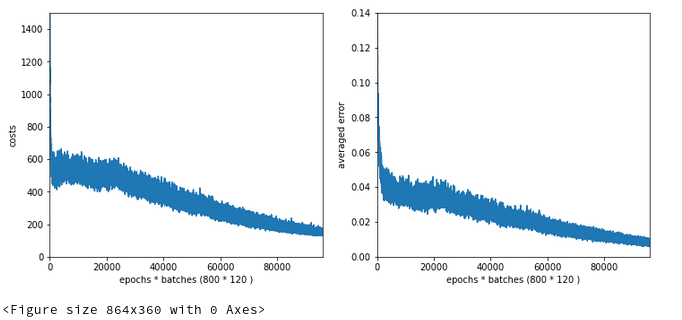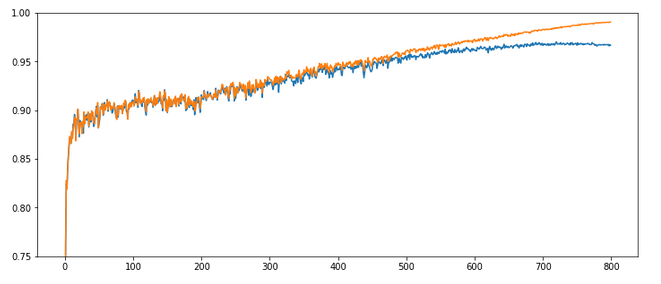The interesting part in this case happens below and at epoch 200: There we see a clear indication that something has „trapped“ us for a while before we could descend into some minimum with the typical split of the accuracy for the training set and the accuracy for the test set. Remember that smaller initial weights also mean an initially smaller contribution of the regularization term to the cost function!

Did we run into a side minimum? Or walk around the edge between two minima? Too complex to analyze in a space with 7000 dimensions!, But, I think this gives you some impression of what might happen on the surface of a varying, bumpy hyperplane …

# Experiment 5: Reduced weights only between the L0/L1 layers

The next test shows the same as the last experiment, but with the initial weights only reduced for the L0/L1 matrix.

Test 5:
Parameters: learn_rate = 0.001, decrease_rate = 0.00001, mom_rate = 0.00005, n_size_mini_batch = 500, Lambda2 = 0.2, n_epochs = 2000, initial weights for the matrix of the first layers L0/L1 within [-0.36 0.36], otherwise in [-0.5, 0.5]:
Results: acc_train: 0.988 , acc_test: 0.967, convergence after ca. 900 epochs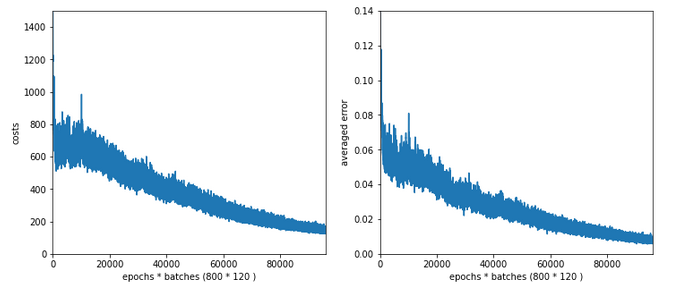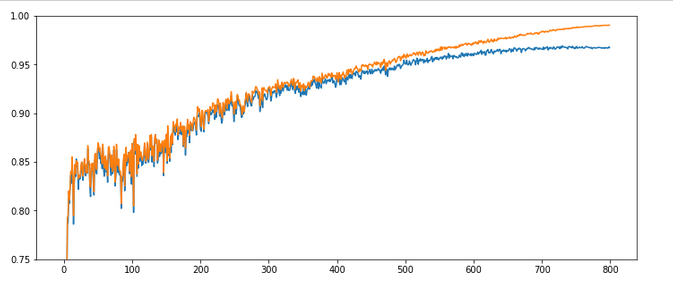All in all – the trouble the code has with finding a way into a global minimum got even more pronounced around epoch 100. It seems as if the algorithm has to find a new path direction there. The lesson learned is: Weight initialization is important!

# Experiment 6: Enlarged mini-batch-size – do we get a smoother evolution?

Now we keep the parameters of experiment 5, but we enlarge the batch size – could be helpful to align and deepen the different minima for the different mini-batches – and thus maybe lead to a smoothing. We choose a batch-size of 1200 (i.e. 50 batches instead of 120 in the training set):

Test 6:
Parameters: learn_rate = 0.001, decrease_rate = 0.00001, mom_rate = 0.00005, n_size_mini_batch = 1200, Lambda2 = 0.2, n_epochs = 2000, initial weights for the matrix first layers L0/L1 [-0.36 0.36], otherwise in [-0.5, 0.5]:
Results: acc_train: 0.959 , acc_test: 0.946, not yet converged after ca. 750 epochs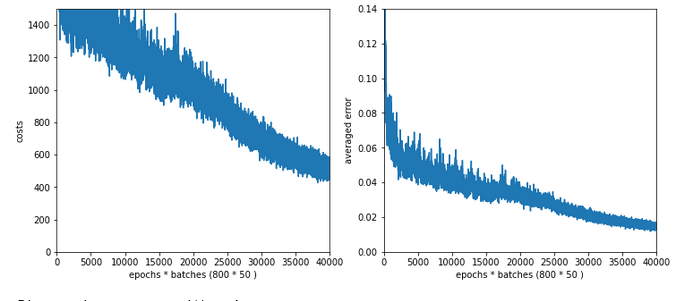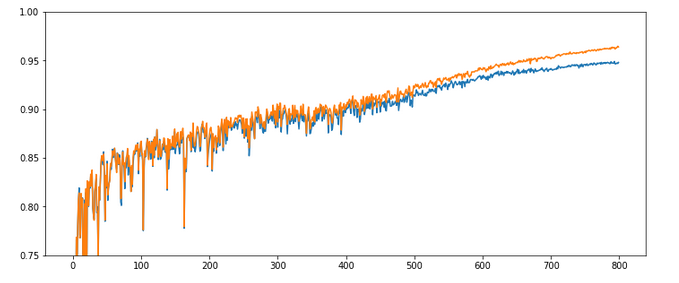Would you say that enlarging the mini-batch-siz really helped us? I would say: Bigger batch-sizes do not help an algorithm on the verge of trouble! Nope, the structural problems do not disappear.

# Experiment 7: Reduced learn-rate, increased decrease-rate

Let us face it: For our present state of the MLP-algorithm and the MNIST X-data values directly fed into the input nodes the learn-rate must be chosen significantly smaller to overcome the initial problems of restructuring the weight matrices. So, we give up our trials to work with larger learn-rates – but only for a moment. Let us for confirmation now reduce the initial learning-rate again, but increase the „decrease rate“. At the same time we also decrease the values of the weights.

Test 7:
Parameters: learn_rate = 0.0002, decrease_rate = 0.00001, mom_rate = 0.00005, n_size_mini_batch = 500, Lambda2 = 0.2, n_epochs = 1200,initial weights for the matrix first layers L0/L1 [-0.36 0.36] and for the next layers L1/L2 + L2/L3 in [0.08, 0.08]:
Results: acc_train: 0.9943 , acc_test: 0.9655, convergence after ca. 600 epochs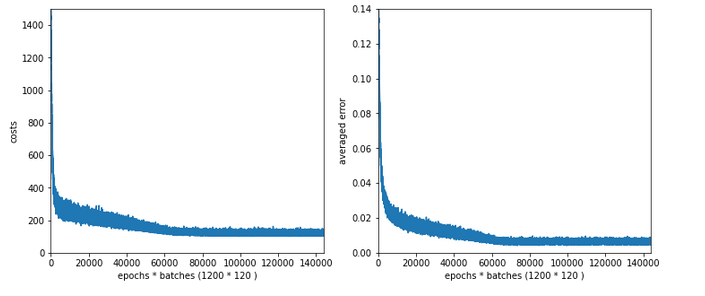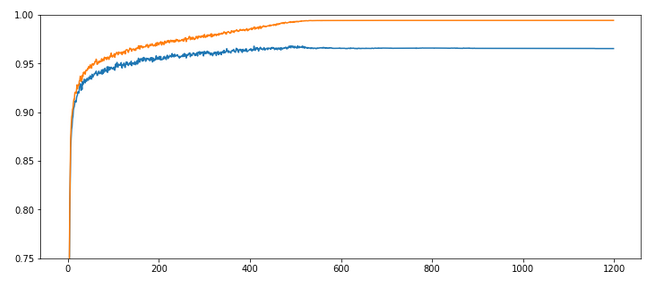OK, nice again! There is some trouble, but we only need 600 epochs to come to a pretty good accuracy value for the test data set!

# Intermediate conclusion

Quite often you may read in literature that a bigger learning rate (often abbreviated with a greek eta) can save computational time in terms of required epochs – as long as convergence is guaranteed. Hmmm – we saw in the tests above that this may not be true under certain conditions. It
is better to say that – depending on the data, the depth of the network and the size of the mini-batches – you may have to control a delicate balance of an initial rate and a rate decline to find an optimum in terms of epochs.

Initial learning rates which are chosen too big together with a too small decrease rate may lead into trouble: the algorithm may get trapped after a few hundred epochs or even stay a long time in some side minimum until it finds a deepening which it really can descent into.

With a smaller learning rate, however, you may find a reasonable path much faster and descent into the minimum much more steadfast and smoothly – in the end requiring remarkably fewer epochs until convergence!

But as we saw with our experiment 4: Even a wiggled start can end up in a pretty good minimum with a really good accuracy. Reducing the learning rate too fast may lead to a circle path with some distance to the minimum. We are talking here about the last < 0.5 percent.

Which minimum level you reach in the end depends on many parameters, but in particular also on the initial weight values. In general setting the initial weight values small enough with respect to the number of nodes on the lower neighbor layer seems to be reasonable.

# The sigmoid function – and a major problem

It is time to think a bit deeper before we start more experiments. All in all one does not get rid of the feeling that something profound is wrong with our algorithm or our setup of the experiments. In my youth I have seen similar things in simulations on non-linear physics – and almost always a basic concept was missing or wrongly applied. Time to care about the math.

An important ingredient in the whole learning via back-propagation was the activation function, which due to its non-linearity has an impact on the gradients which we need to calculate. The sigmoid function is a smooth function; but it has some properties which obviously can lead to trouble.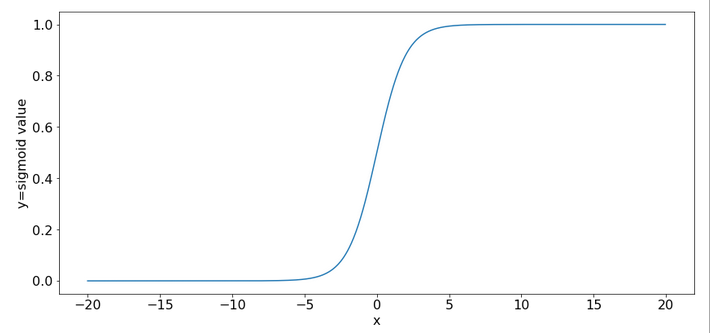One is that it produces function values pretty close to 1 for arguments x > 15.

```sig(10) = 0.9999546021312976
sig(12) = 0.9999938558253978
sig(15) = 0.9999998874648379
sig(20) = 0.9999999979388463
sig(25) = 0.9999999999948910
sig(30) = 0.9999999999999065
```

So, function values for bigger arguments can almost not be distinguished and resulting gradients during backward propagation will get extremely small. Keeping this in mind we turn towards the initial steps of forward propagation. What happens to our input data there?

We directly present the feature values of the MNIST data images at 784 input nodes in layer L0. The following sketch only shows the basic architecture ofa a MLP; the node numbers do NOT correspond to our present MLP.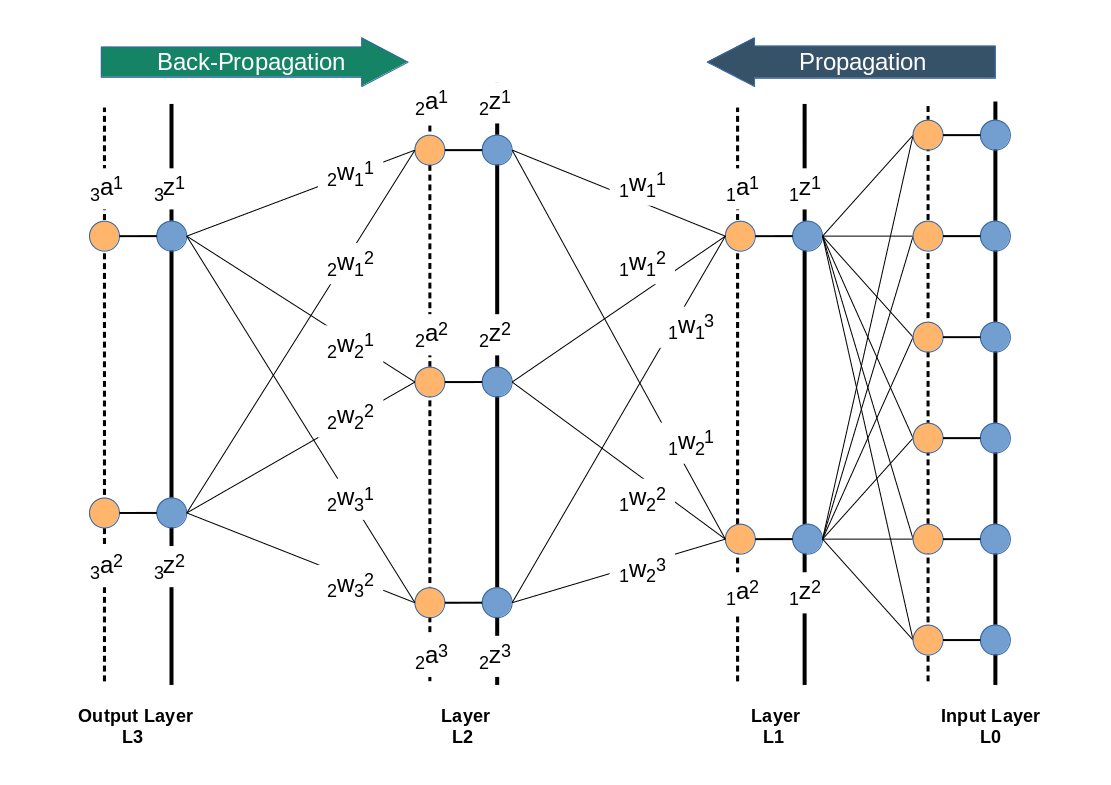Then we multiply by the weights (randomly chosen initially from some interval) and accumulate 784 contributions at each of the 70 nodes of layer L1. Even if we choose the initial weight values to be in range of [-0.5, +0.5] this will potentially lead to big input values at layer L1 due to summing up all contributions. Thus at the output side of layer L1 our sigmoid function will produce many almost indistinguishable values and pretty small gradients in the first steps. This is certainly not good for a clear adjustment of weights during backward propagation.

There are two remedies, one can think about:

• We should adapt the initial weight values to the number of nodes of the lower
layer in forward propagation direction. A first guess would be something in the range 1.0e-3 for weights between layer L0 and L1 – assuming that ca. 10% of the 784 input features show values around 220. Weights between layers L1 and L2 should be in the range of [-0.05, 0.05] and between layer L2 and L3 in the range [-0.1, 0.1] to prevent maximum values above 5.
• We should scale down the input data, i.e. we should normalize them such that they cover a reasonable value range which leads to distinguishable output values of the sigmoid function.

A plot for the first option with a reasonably small learn-rate as in experiment 7 and weights following the 1/sqrt(num_nodes) at every layer (!) is the following :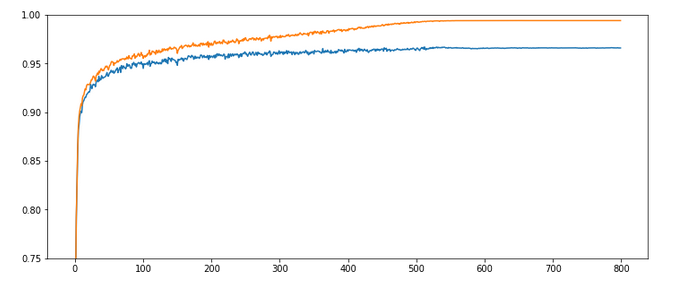Quite OK, but not a breakthrough. So, let us look at normalization.

# Normalization – Standardization

There are different methods how one can normalize values for a bunch of instances in a set. One basic method is to subtract the minimum value „x_min“ of all instances from the value of each instance followed by a division of the difference between the max value (x_max) and the minimum value (x_max – x_min): x => (x – x_min) / (x_max – x_min).

A more clever version – which is called „standardization“ – subtracts the mean value „x_mean“ of all instances and divides by the standard deviation of the set. The resulting values have a mean of zero and a variance of 1. The advantage of this normalization approach is that it does not react strongly to extreme data values in the set; still it reduces big values to a very moderate scale.

SciKit-Learn provides the second normalization variant as a function with the name „StandardScaler“ – this is the reason why we introduced an import statement for this function at the top of this article.

# Code modifications to address standardization of the input data

Let us include standardization in our method to handle the input data:

Modifications to function „_method _handle_input_data()“:

```    ''' -- Method to handle different types of input data sets --'''
def _handle_input_data(self):
'''
Method to deal with the input data:
- check if we have a known data set ("mnist" so far)
- reshape as required
- analyze dimensions and extract the feature dimension(s)
'''
# check for known dataset
try:
if (self._my_data_set not in self._input_data_sets ):
raise ValueError
except ValueError:
print("The requested input data" + self._my_data_set + " is not known!" )
sys.exit()

# MNIST datasets
# **************

# handle the mnist original dataset - is not supported any more
#if ( self._my_data_set == "mnist"):
#    mnist = fetch_mldata('MNIST original')
#    self._X, self._y = mnist["data"], mnist["target"]
#    print("Input data for dataset " + self._my_data_set + " : \n" + "Original shape of X = " + str(self._X.shape) +
#      "\n" + "Original shape of y = " + str(self._y.shape))
#
# handle the mnist_784 dataset
if ( self._my_data_set == "mnist_784"):
mnist2 = fetch_openml('mnist_784', version=1, cache=True, data_home='~/scikit_learn_data')
self._X, self._y = mnist2["data"], mnist2["
target"]
print ("data fetched")
# the target categories are given as strings not integers
self._y = np.array([int(i) for i in self._y])
print ("data modified")
print("Input data for dataset " + self._my_data_set + " : \n" + "Original shape of X = " + str(self._X.shape) +
"\n" + "Original shape of y = " + str(self._y.shape))

# handle the mnist_keras dataset
if ( self._my_data_set == "mnist_keras"):
(X_train, y_train), (X_test, y_test) = kmnist.load_data()
len_train =  X_train.shape
len_test  =  X_test.shape
X_train = X_train.reshape(len_train, 28*28)
X_test  = X_test.reshape(len_test, 28*28)

# Concatenation required due to possible later normalization of all data
self._X = np.concatenate((X_train, X_test), axis=0)
self._y = np.concatenate((y_train, y_test), axis=0)
print("Input data for dataset " + self._my_data_set + " : \n" + "Original shape of X = " + str(self._X.shape) +
"\n" + "Original shape of y = " + str(self._y.shape))
#
# common MNIST handling
if ( self._my_data_set == "mnist" or self._my_data_set == "mnist_784" or self._my_data_set == "mnist_keras" ):
self._common_handling_of_mnist()

# handle IMPORTED datasets
# **************************+
if ( self._my_data_set == "imported"):
if (self._X_import is not None) and (self._y_import is not None):
self._X = self._X_import
self._y = self._y_import
else:
print("Shall handle imported datasets - but they are not defined")
sysexit()
#
# number of total records in X, y
# *******************************
self._dim_X = self._X.shape

# Give control to preprocessing - has to happen before normalizing and splitting
# ****************************
self._preprocess_input_data()
#
# Common dataset handling
# ************************
# normalization
if self._b_normalize_X:
# normalization by sklearn.preprocessing.StandardScaler
scaler = StandardScaler()
self._X = scaler.fit_transform(self._X)

# mixing the training indices - MUST happen BEFORE encoding
shuffled_index = np.random.permutation(self._dim_X)
self._X, self._y = self._X[shuffled_index], self._y[shuffled_index]

# Splitting into training and test datasets
if self._num_test_records > 0.25 * self._dim_X:
print("\nNumber of test records bigger than 25% of available data. Too big, we stop." )
sysexit()
else:
num_sep = self._dim_X - self._num_test_records
self._X_train, self._X_test, self._y_train, self._y_test = self._X[:num_sep], self._X[num_sep:], self._y[:num_sep], self._y[num_sep:]

# numbers, dimensions
self._dim_sets = self._y_train.shape
self._dim_features = self._X_train.shape
print("\nFinal dimensions of training and test datasets of type " + self._my_data_set +
" : \n" + "Shape of X_train = " + str(self._X_train.shape) +
"\n" + "Shape of y_train = " + str(self._y_train.shape) +
"\n" + "Shape of X_test = " + str(self._X_test.shape) +
"\n" + "Shape of y_test = " + str(self._y_test.shape)
)
print("\nWe have " + str(self._dim_sets) + " data records for training")
print("Feature dimension is " + str(self._dim_features))

# encoding the y-values = categories // MUST happen AFTER encoding
self._get_num_labels()
self._encode_all_y_labels(self._b_print_test_data)
#
''' Remark: Other input data sets can not yet be handled '''
return None
#
```

Well, this looks a bit different compared to our original function. Actually, we perform normalization twice. Once inside the new function „_preprocess_input_data()“ and once afterwards.

New function „_preprocess_data()“:

```'''----------
Method to preprocess the input data
----------------------------------- '''
def _preprocess_input_data(self):

if self._b_normalize_X:
# normalization by sklearn.preprocessing.StandardScaler
scaler = StandardScaler()
self._X = scaler.fit_transform(self._X)

# Clustering
if self._b_perform_clustering:
self._perform_clustering()
print("\nClustering started")
else:
print("\nNo Clustering requested")

return None
#
```

The reason is that we have to take into account other transformations of the input data by other methods, too. One of these methods will be clustering, which we shall investigate in a forthcoming article. (For the nervous ones among the readers: The StandardScaler is intelligent enough to avoid divisions by zero means at the second time it is called!)

# Experiment 8: Standardizes input data, reduced learn-rate, increased decrease-rate and „1/sqrt(nodes)-rule for the initial weights of all layers

We shall call our class My_ANN now with the parameter „b_normalize_X = True“, i.e. we standardize the whole MNIST input data set X before we split it into a training and a test data set.

In addition we apply the rule to set the interval-borders for initial weights to [-1.0/sqrt(num_nodes_layer), 1.0/sqrt(num_nodes_layer)], with „num_nodes_layer“ being the number of nodes in the lower layer which the weights act upon during forward propagation.

Test 8:
Parameters: learn_rate = 0.0002, decrease_rate = 0.00001, mom_rate = 0.00005, n_size_mini_batch = 500, Lambda2 = 0.2, n_epochs = 2000, weights at all layers in [- 1.0/sqrt(num_nodes_layer), 1.0/sqrt(num_nodes_layer)]
Results: acc_train: 0.9913 , acc_test: 0.9689, convergence after ca. 650 epochs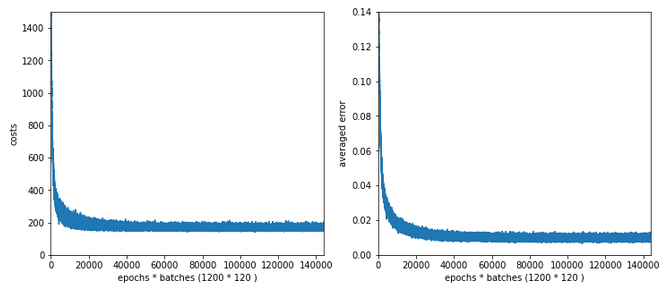Wow, extremely smooth curves now – and we got the highest accuracy so far!

# Experiment 9: Standardized input, bigger initial learning rate, enlarged intervals for weight initialization

We get brave again! we enlarge the learning-rate back to 0.001. In addition we enlarge the intervals for a random distribution of initial weights for each layer by a factor of 2 =>- [-2*1.0/sqrt(num_nodes_layer), 2*1.0/sqrt(num_nodes_layer)].

Test 9:
Parameters: learn_rate = 0.001, decrease_rate = 0.00001, mom_rate = 0.00005, n_size_mini_batch = 500, n_epochs = 1200, weights at all layers in [-2*1.0/sqrt(num_nodes_layer), 2*1.
0/sqrt(num_nodes_layer)]
Results: acc_train: 0.9949 , acc_test: 0.9754, convergence after ca. 550-600 epochs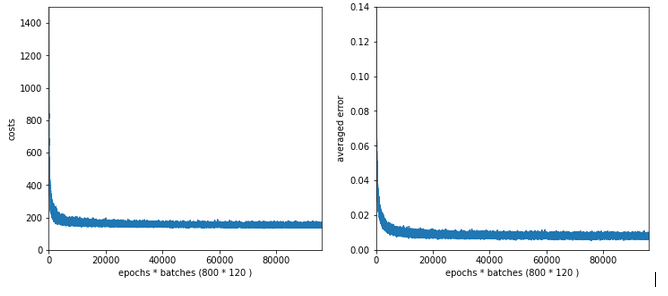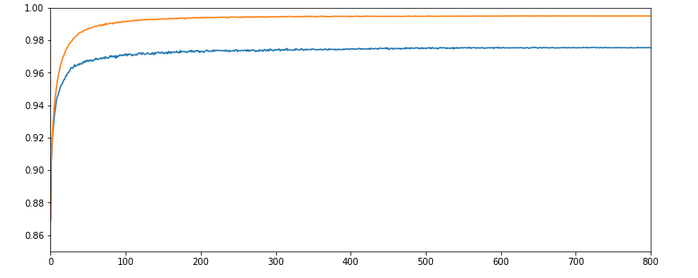Not such smooth curves as in the previous plot. But WoW again – now we broke the 0.97-threshold – already at an epoch as small as 100!

I admit that a very balanced initial statistical distribution of digit images across the training and the test datasets helped in this specific test run, but only a bit. You will easily and regularly pass a value of 0.972 for the accuracy on the test dataset during multiple consecutive runs. Compared to our reference value of 0.96 this is a solid improvement!

But what is really convincing is the fact the even with a relatively high initial learning rate we see no major trouble on our way to the minimum! I would call this a breakthrough!

# Conclusion

We learned today that working with mini-batch training can be tricky. In some cases we may need to control a balance between a sufficiently small initial learning rate and a reasonable reduction rate during training. We also saw that it is helpful to get some control over the weight initialization. The rule to create randomly distributed initial weight values initialization within intervals given by [n*1/sqrt(num_nodes), n*1/sqrt(num_nodes)] appears to be useful.

However, the real lesson of our experiments was that we do our MLP learning algorithm a major favor by normalizing and centering the input data.

At least if the sigmoid function is applied as the activation function at the MLP’s nodes a initial standardization of the input data should always be tested and compared to training runs without standardization.

In the next article of this series

A simple Python program for an ANN to cover the MNIST dataset – XIII – the impact of regularization

we shall have a look at the impact of the regularization parameter Lambda2, which we kept constant, so far. An interesting question in this context is: How does the ratio between the (quadratic) regularization term and the standard cost term in our total loss function change during a training run?

In a further article to come we will then look at a method to detect clusters in the feature parameter space and use related information for gradient descent. The objective of such a step is the reduction of input features and input nodes. Stay tuned!